Next: Specification of Potentials Up: OPTIM User Guide Previous: The odata file   Contents

# Keywords

The following keywords are recognised, where n, x and exec are integer, real and character data, respectively.

• 2D: restrict the system to the x - y plane.

• AAORIENT k sigma: adds a mean field to the general angle-axis potential. The strength of the field is determined by the parameter k (default 1). Positive k values will tend to align the reference frames of all the rigid bodies, while negative k values penalise alignment. The field is designed to fix geometry optimisation problems caused by rigid body rotations that give very small Hessian eigenvalues. The zero eigenvalues should be shifted roughly in proportion to k. If range is specified, the field is modulated by a Gaussian with standard deviation range, to allow local alignment while removing the contributions from distant rigid bodies.

• ACE n: for use with CHARMM runs that employ the ACE implicit solvent model. n specifies the number of calls to the CHARMM energy and gradient for which lists are held fixed. The default value of n is 50. Note: ENDNUMHESS must be used with the ACE solvent model. Otherwise OPTIM will attempt to use incorrect second derivatives. ENDNUMHESS will be needed in the odata.start, odata.finish and odata.connect files. For odata.start and odata.finish the keyword ENDHESS must also be included.

• ACKLAND id: specifies an Ackland embedded atom metal potential coded by Dr Mihai-Cosmin Marinica. id specifies the particular metal: 5 is iron, 6 is a different iron, 7 is tungsten. Positive values for id specify periodic boundary conditions, where box lengths must be specified by the PERIODIC keyword. See also ACKLAND1 and ACKLAND2.

• ACKLAND1 : specifies the original Ackland embedded atom metal potential coded by Dr Mihai-Cosmin Marinica. The ACKLAND keyword is also needed.

• ACKLAND2 : specifies the new Ackland embedded atom metal potential coded by Dr Mihai-Cosmin Marinica. The ACKLAND keyword is also needed.

• ADJUSTK frq tol frac: causes the force constant to be adjusted dynamically in doubly-nudged elastic band runs. frq is the frequency at which the adjustment is made, based on the deviation of the image spacing from uniformity. If the spacing deviates by more than tol then the force constant is increased by frac; if it is lower then the force constant is decreased by frac.

• ADM n: will cause the interatomic distance matrix to be printed every n cycles; the default for n is 20. This matrix is not printed by default.

• ALIGNRBS n1, n2, ...: For use with GENRIGID. Specify that the translation and rotation used for endpoint alignment should be calculated from a subset of rigid bodies in the system, specified by n1, n2, .... The number of arguments that may be specified is limited by the allowed length of the keyword line. The calculated translation and rotation are applied to the whole system. Note, there is currently no permutational alignment implemented, so this routine will not detect permutational isomers.

• ALPHA: sets exponent value for averaged Gaussian and Morse potentials, default value is 6.

• ALLPOINTS: turns on printing of coordinates to file points for all intermediate configurations. Default is false.

• AMBER12 inpcrd inpcrdformat: specifies calculation with the interfaced version of the AMBER 12 pmemd program. AMBER 12 requires an additional input file, min.in, which specifies keywords for the AMBER 12 potential. It also requires appropriate topology and coordinates, in files named coords.prmtop and coords.inpcrd respectively. For details, see the AMBER 12 manual. As with the AMBER 9 interface, cutoffs are smoothed, using the min.in keyword ifswitch=1. Additional keywords are as AMBER 9, though it should be noted that analytical second derivatives are not available through use of the NAB keyword. Users of GMIN should note that, unlike in GMIN, specifying AMBER12 without inpcrdformat assumes that input coordinates are provided in xyz, rather than AMBER restart format.

• A12DECOMPE: dumps out decomposed energy contribution at the end of optimisations.
• A12DECOMPEALL dumps the decomposition for every call to potential.

• AMBER9 inpcrd inpcrdformat: specifies a calculation with the interfaced version of the Amber 9 program package. From this package the Amber force fields are being used, with small modifications (e.g. smooth cut-offs). Starting coordinates do not need to be specified in the odata file, they are read from inpcrd instead (default coords.inpcrd), in Amber inpcrd file format specified by the second optional argument inpcrdformat. If the second argument is missing, it is assumed that inpcrd contains only three columns with the xyz coordinates of all atoms, in the same order as in the topology file. To start a run with this interface, several auxiliary files are required in the same directory: input coordinate file coords.inpcrd, parameter topology file coords.prmtop, input file to Amber containing force field specifications min.in, and, if desired, a coordinate file different from coords.inpcrd containing starting coordinates. To turn on smooth cutoffs for the Generalised Born force fields, the keyword ifswitch=1 has to be used in the &cntrl namelist block of min.in. When using the AMBER9 keyword, any calculated second derivatives will be numerical. If one wants analytical second derivatives, the NAB keyword should be used instead, with the same syntax. The NAB interface does not currently support smooth cutoffs, so analytical second derivatives are only recommended for stationary points calculated with large cutoffs. Additional keywords for the AMBER 9 runs are NAB and DUMPSTRUCTURES.

• AMBERIC: interpolation in bhinterp with internal coordinates. If BACKBONE specified additionally (AMBERIC BACKBONE) the protein backbone is also interpolated in internals. Does not work for polymers.

• AMBERSTEP: perturbation/twisting in bhinterp done with internal coordinates

• AMBPERTONLY n:only perturb angles for which the difference between the start and end configuration before the interpolation was greater than n (i.e. n= 30.0 - 30 degree). Probability of perturbation depends on this likelihood (and on position in chain)

• AMBPERTOLD: use original perturbation scheme. Now angles depend on position in chain - larger perturbation if at the end.

• ANGLEAXIS: specifies angle/axis coordinates for rigid body TIP potentials.

• ARCTOL tol: specifies the accuracy tolerance for computing the inverse arc length used in the cubic spline interpolated string methods for double-ended transition state searches. The default is 10-4.

• ATOMMATCHDIST: used for bulk systems, providing an alternative method for finding the shortest distance between structures. This method is particularly useful for finding the shortest distance between very similar structures such as defective crystal structures. The method is an extension of the original bulkmindist.f90 routine for matching permutationl isomers. Atoms are overlayed and the number of exactly matching g atoms is maximised. (ATOMMATCHINIT uses atom matching only for aligning initial structures and then reverts to the default distance method.) With ATOMMATCHDIST/INIT, the method is non-deterministic to maximise efficiency. It has been optimised to work particularly well for providing small distan ces between similar structures and for removing very large distances. However, it will not always outperform the default method for middling distances. Currently only one method for calculating distances, atom matching or the default, can be used.

• ATOMMATCHFULL: As for ATOMMATCHDIST, but provides a slow deterministic result that should always outperform or equal the default distance calculation and ATOMMATCHDIST but may never finish.

• ATOMMATCHINIT: see ATOMMATCHDIST.

• AUCMLPLOG: Logical, if included, a log function is used as loss function instead of AUC. This keyword makes the AUCMLPVB3 routine equivalent to the original MLPVB3 routine. Default is FALSE.

• AUCMLPVB3 in hidden out data λ β: Specifies a single-layered neural network as with keyword MLPVB3 (see below), with in input nodes, hidden hidden nodes, out output nodes and λ as the L2 regularisation parameter. data is the number of data points the model trains with, stored in a file called MLPData. This file should be formatted as follows:  y1 x11 x21 x31 ... y2 x12 x22 x32 ... y3 x13 x23 x33 ... ... yNdata x1Ndata x2Ndata x3Ndata ...
where yi is the outcome for data point i, and xji is the jth input feature for data point i. The features will be read starting from the feature in column start (ignoring the y column).

Unlike MLPVB3, instead of calculating the associated loss value, an approximated AUC is calculated. The true AUC value is given by the following non-differentiable function:

 AUC(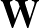;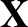) =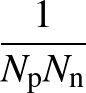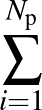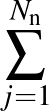Θ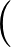p1(;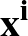) - p1(;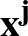)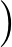, (1)

where Θ(x) is the Heaviside step function (1 for x > 0 and 0 otherwise). This function is not differentiable, so we must use an approximate function. The function to be minimised is

 E(;) =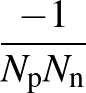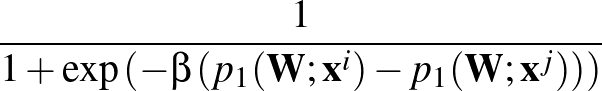. (2)

p1(;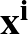) is the softmax probability of the neural network giving output class 1 for data point i, given weights. The sums are over data points where the true class is 1, of which there are Np, and over data points where the true class is not 1, of which there are Nn. β is the parameter for the sigmoid function

 σ(z)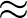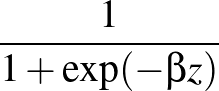. (3)

Larger values of β make curve steeper, hence approximate the true AUC more closely. The maximum value allowed for β is set at 500 to prevent floating-point underflow errors. If β is set higher than this value, the maximum value of 500 will override it and be used instead.

• AVOIDCOLLISIONS tol: For use with GENRIGID. When performing the angle-axis interpolation prior to a DNEB run, check the energy of the highest-energy image. If it is greater than tol, then for each rigid body in turn we reverse the sense of interpolation for its angle axis vector (so the body rotates in the opposite direction to before). Hopefully this will avoid two rigid bodies colliding (which is assumed to be what causes the high-energy image). If reversing the rotation direction results in a decreased maximum image energy, the new sense of rotation is accepted. If at any point the maximum image energy falls below tol, the testing process is stopped.

This is currently a rather crude method and not terrible effective. It will be improved in the future.

• AXIS n: specifies the highest symmetry axis to search for in routine symmetry; default is six.

• BBCART: use Cartesian coordinates for all backbone atoms and for prolines. Right now, only works for natural internals.

• BBRSDM gamma epsilon sigma1 sigma2 M alpha gmax nstep: specifies the steepest-descent minimiser introduced by Barzilai and Borwein  and modified by Raydan  for a maximum of nstep iterations in a pathway calculation. The method uses gradient only information with the convergence criterion gmax for the RMS force and does not guarantee descent in the objective function in each iteration. The input parameters include an integer M≥ 0, γ∈(0, 1), 0 < σ1 < σ2 < 1, and an initial value for α. Raydan reported with γ = 10-4, ε = 10-10, σ1 = 0.1, σ2 = 0.5, α0 = 1.0, and M = 10.

• BFGSCONV gmax: gmax is the convergence criterion for the root-mean-square gradient, default 0.001. This is also the convergence criterion for the subspace minimisations in hybrid EF/BFGS transition state searches, for use with BFGSTS.

• BFGSMIN gmax: instructs the program to perform an LBFGS minimisation. gmax is the convergence criterion for the root-mean-square gradient, default 0.001.

• BFGSSTEP: instructs the program to step off a saddle point along the eigenvector corresponding to the smallest negative eigenvalue without diagonalizing the Hessian to find this eigenvector. It is possible to step off parallel and antiparallel to this eigenvector by specifying positive or negative values for the MODE parameter. BFGSTS need not be set, but see also PATH and CONNECT. After taking the first step the program switches to energy minimisation using whichever algorithm is specified, e.g. BFGSMIN, SEARCH 0, SEARCH 6, RKMIN, BSMIN, etc. It should no longer be necessary to use this keyword, as OPTIM will now perform a pathway calculation after a single-ended transition state search if the PATH keyword is present. The minimiser for the pathway phase can be chosen independently of the transition state search algorithm by specifying one of the BFGSMIN, BSMIN, RKMIN, BBRSDM, or PMPATH keywords.

• BFGSSTEPS n: sets the maximum number of steps allowed in LBFGS minimisations. Default value is the value of the STEPS parameter. However, for NEWNEB this parameter is specified via the NEWCONNECT or NEWNEB line.

• BFGSTS nevs ncgmax1 ncgmax2 ceig nevl: instructs the program to perform a hybrid BFGS/eigenvector-following transition state search. nevs is an integer that defines the largest number of iterations allowed in the calculation of the smallest Hessian eigenvalue; default 500. ncgmax1 is an integer that defines the largest number of BFGS steps allowed in the subspace minimisation before the eigenvalue is deemed to have converged; default 10. ncgmax2 is an integer that defines the largest number of BFGS steps allowed in the subspace minimisation after the eigenvalue is deemed to have converged; default 100. This parameter is ignored if NOIT is set. The eigenvalue is deemed to be converged if the modulus of the overlap between the corresponding eigenvector and that saved from the previous step exceeds 0.999 and we have the right number of negative eigenvalues. ceig is a double precision parameter that sets the convergence criterion for the calculation of the smallest non-zero Hessian eigenvalue. If NOHESS is set ceig is compared to the RMS force' corresponding to the Rayleigh-Ritz expectation value that is minimised to get the smallest eigenvalue. If the Hessian is used via iteration or NOIT then ceig is compared to the percentage change in the eigenvalue between successive steps. The default value is 1.0, which is more appropriate to the latter case. Smaller values are probably necessary in BFGS/BFGS calculations. nevl is an integer that defines the largest number of iterations allowed in the calculation of the largest Hessian eigenvalue; default 100. Not needed if NOHESS of NOIT is set.

• BFGSTSTOL tol: tolerance for eigenvector overlap in BFGSTS where the number of tangent space steps switches from small to large. If the overlap differs from unity by less than tol then the larger number of steps is used in the tangent space minimisation. 0.0001 is the default value.

• BFGSTSPC evpc: convergence condition for absolute value of percentage change in the Hessian eigenvalue calculated using the Rayleigh-Ritz procedure for transition state searches with no Hessian. Default value is 50%. This condition complements the tolerance parameter ceig of BFGSTS above. It can prevent premature convergence when an eigenvalue estimate passes through zero, where the RMS force' can be small and accidentally satisfy the condition on ceig.

• BFGS: specifies that the full BFGS minimiser should be used. Inefficient compared to LBFGS.

• BGUPTAT NTYPEA AAA PAA QAA ZAA R0AA: One of the required keywords to specify a Binary Gupta run. NTYPEA is specified, followed by the potential parameters for the A-A interactions. See also BGUPTATAB and BGUPTATBB. The atom coordinates in odata must also be labelled by G1 and G2 (representing A and B type atoms respectively). All the G1 atoms must be first in the file, followed by the G2 atoms (the calculation is performed assuming the coordinates are of NTYPEA A atoms first, and the coordinates listed afterwards are B).

• BGUPTATAB AAB PAB QAB ZAB R0AB: The line to specify the potential parameters for the A-B interactions for a Binary Gupta run.

• BGUPTATBB ABB PBB QBB ZBB R0BB: Specifies the B-B interaction parameters.

• BHDEBUG : printing localised debugging output for BHINTERP

• BHINTERP dthresh maxe bhsteps conv T stepsize accrat K sfrac ICINTERP: interpolation between minima in double-ended searches using CONNECT is changed to search for likely intermediate minima. The interpolation is performed for pairs of minima separated by a minimum distance more than dthresh. Intermediate minima are only accepted if their energy is below maxe. A basin-hopping global optimisation run of bhsteps is run for each pair of end point minima within dthresh using an RMS gradient convergence criterion of conv, a temperature parameter of T, and a maximum step size for perturbations of stepsize. The step size is adjusted dynamically towards an acceptance ratio target of accrat. The objective function consists of the energy of the minimum on the potential energy surface plus the energy corresponding to harmonic springs of force constant K stretched to displacements corresponding to the minimum distance between the new minimum and the end points. sfrac is used in the initial interpolation: a value of 0.5 will put the initial guess half-way between the end points, and in general the geometry will be sfrac times one end point plus (1 -sfrac) times the other. For large distances, using a value other than a half may be helpful. If ICINTERP is present then amino acid side chains are interpolated using internal coordinates for CHARMM runs. The step size is interpreted in degrees for CHARMM, where the perturbations used to step between minima are performed using dihedral angle twists. The algorithm is applied recursively between minima as new minima are found. A new minimum will not be accepted if both distances to the minima we are currently trying to interpolate between are greater than the minimum distance between these minima.

• BHINTERPUSELOWEST CHECKENER bhstepsmin: take the minimum with the lowest potential energy as the best intervening structure, rather than the one with the lowest potential energy plus spring energy. The accept/reject procedure in the BHINTERP procedure is still based on the potential energy plus spring energy. CHECKENER is an optional argument. If it is set, for each accepted BHSTEP it is checked whether the potential energy of the best intervening structure is smaller than maxe, which is determined together with the BHINTERP keyword. If so, and if at least bhstepsmin BHINTERP steps have been executed, the BHINTERP run for the current pair of endpoints is stopped. This procedure ensures some dynamic adjustment of BHINTERP to the distance between the two endpoints in question. If CHECKENER is not given, BHINTERP performs bhsteps steps (see above). bhstepsmin is an optional argument, whose default is 1.

• BINARY ntypea epsab epsbb sigmaab sigmabb: specifies a binary Lennard-Jones system for use with the LP or LS atom types. ntypea is the number of type A atoms—the rest are assumed to be type B and appear at the end of the list of coordinates. εAA = σAA = 1 define the units of energy and length, and epsab= εAB, epsbb= εBB, sigmaab= σAB, sigmabb= σBB.

• BISECT dthresh maxe bisectsteps attempts ICINTERP: specifies the BISECT interpolation option, where no transition state searches are run. This option is intended for use with DUMMYTS in PATHSAMPLE. The distance threshold parameter specifies that bisection between consecutive minima should occur if their minimum distance is greater than dthresh. The interpolated geometry is minimised and compared with the starting structures. New minima are only accepted if their energy is below maxe; they are added to the current list of known minima between the two starting structures originally supplied to OPTIM. bisectsteps is the maximum number of bisection steps, and attempts is the number of attempts per step for a given pair. If minimisation leads to one of the end points the interpolation is changed to use fractions of the two structures that become increasingly skewed, as for the adjustment of sfrac with BHINTERP, above. If ICINTERP is present then amino acid side chains are interpolated using internal coordinates for CHARMM runs.

• BLN rkr rkt: general BLN protein bead model.[21,22] rkr and rkt are the spring constants for bond length and bond angle terms. An auxiliary file BLNsequence is required to specify all the other parameters and the sequence, along with a code to define the secondary structure template that changes some of the parameters. For example, the format of BLNsequence for protein L is: comment: LJREP_BLN >0 and LJATT_BLN <0 for B-B, L-L and L-B, N-L and N-B and N-N 1.0D0 -1.0D0 0.33333333333333D0 0.33333333333333D0 1.0D0 0.0D0 comment: coefficients A, B, C, D of 1 + cosφ, 1 - cosφ, 1 + cos 3φ and 1 + cos(φ + π/4) comment: for Helical, Extended and Turn residues in order, four per line 0.0D0 1.2D0 1.2D0 1.2D0 0.9D0 0.0D0 1.2D0 0.0D0 0.0D0 0.0D0 0.2D0 0.0D0 LBLBLBLBBNNNBBBLBLBBBNNNLLBLLBBLLBNBLBLBLBLNNNLBBLBLBBBL EEEEEETEHTHEEEEEEEEHHEHHHHHHHHHHEHTEEEEEEETTTEEEEEEEE

• BLNGO kr kθ λ: specifies a Gō potential with the same form as the BLN potential. The parameters kr and kθ are the same as those used for the BLN keyword and a BLNsequence file is required. Also needed is an auxiliary file, contactmap, containing the pairs of residues in contact in the native state of the protein with one pair of residue numbers in each line of the file. An optional parameter, λ, specifies the strength of the non-native interactions in a scaled BLN potential .

• BOND a1 a2: Specifies an extra bond to add to the structure when using internal coordinates. Adding a bond between ligand and protein allows the generation of a complete set of natural internal coordinates. The bond should be between two atoms numbered relatively close together to avoid KD getting too large. The recommended method of dealing with a ligand is still to use Cartesian coordinates for it with CARTRESSTART instead.

• BOWMAN n path: specifies one of the Bowman potentials for water. n is 2 or 3, where 2 is the full Bowman potential and 3 is the so-called Kumar-Skinner 3-body expansion, which is faster, but less accurate. path is the location of the various data files coef-3b/&sstarf#star; called by the potential. The file h4o2.pes4.coeff.dat must be present in the current working directory, and the atom order must be H1a, H1b, H2a, H2b,..., O1, O2,... The energy is in hartree and the distance is in Angstrom.

• BOXDERIV boxlx boxly boxlz alpha beta gamma: specifies optimisation of the cell parameters for periodic systems. No argument is needed for if the cell parameters are otherwise known e.g. read from dftb_in.hsd in a DFTBP setup, or from the system.cell file for CASTEP, or the POSCAR file for VASP. For CASTEP and VASP the BOXDERIV directive also sets USEFRAC so that the atomic coordinates are manipulated in fractional form during the optimisation. Otherwise, boxlx, boxly, boxlz, alpha, beta, gamma are the box lengths and angles, in radians, of the initial structure. If only the box lengths are given, the cell will be taken as orthorhombic, and only the cell lengths will be optimized. The cell lengths and angles of the initial structure should also be specified using the PERIODIC keyword. If the gradients wrt cell parameters are being calculated for a system using atomic, Cartesian coordinates, the initial coordinates in the coords file should be in fractional coordinates. If the gradients wrt cell parameters are being calculated for a system using rigid body coordinates, the initial coordinates coordinates should be given in the usual way for GENRIGID, and the RIGIDINIT keyword should be used.

• BOXSTEP boxstepfreq: for use with the framework for optimizing cell parameters using the BOXDERIV keyword. Randomly changes the cell lengths and angles every boxstepfreq basin-hopping stes.

• BOXPRESSURE pressure: for use with variable cells and the BOXDERIV keyword. Uniformly applies pressure GPa to the cell via the stress tensor. A corresponding p*V term is added to the energy, making it the enthalpy.

• BSMIN gmax eps: calculates a steepest-descent path using gradient only information with convergence criterion gmax for the RMS force and initial precision eps. The Bulirsch-Stoer algorithm is used.

• BULK boxx boxy boxz: specifies that a periodically repeated supercell is being used. The parameters boxx, boxy, and boxz specify the dimenions of the rectangular box. Note that many potentials specify box dimensions using the PARAMS keyword. When using RIGIDINIT, specify box dimensions using BULK as well as with PARAMS.

• CADPAC system exec: tells the program to read derivative information in CADPAC format. system is a string to identify the system and exec is the name of an executable that will generate a CADPAC input deck from a points file. If exec is omitted its name is assumed to be editit.system.

• CALCDIHE: calculates an order parameter for CHARMM and UNRES with respect to a reference structure in file ref.crd.

• CALCRATES temp h: rate constants will be calculated for each transition state found in a CONNECT or PATH run, or using saved information in an old path.info file if READPATH is specified. Both forward and backward canonical rate constants are calculated from transition state theory at temperature temp (default temp=1), and values are given for both quantum and classical harmonic vibrational partition functions. h is the value of Planck's constant in suitable reduced units for the corresponding potential (default value 1).

• CAMSHIFT csversion svnroot shiftfile csn csalpha: uses chemical shifts as restraints during the optimization procedure. Currently, CAMSHIFT can only be used together with CHARMM. csversion is a string that specifies the method for combining the two potentials: MERGE means (1 - α)*Charmm + α*Camshift, ORIGINAL means Charmm + α*Camshift, and NOFF means α*Camshift. svnroot specifies the svn root directory (e.g., HOME/svn/). shiftfile is the file containing the experimental shifts, which has to be located in the working directory. csversion, svnroot and shiftfile all have to be specified together with CAMSHIFT. csn and csalpha are optional parameters. They define the tolerance parameter of the CamShift energy profile, and the relation between CamShift and the force field, respectively. Default values for both are 1.0. • CANDIDATES value: specifies which images in an NEB interpolation should be used as starting points for transition state searches. value can be high' (just the highest image) or maxim' (all local maxima, the default). • CAPSID rho eps r h: specifies a potential for rigid pentagonal pyramids with Morse parameter rho, repulsive strength eps, radius r, and height h (in units of r). h may be omitted, in which case it defaults to 0.5. • CARTRESSTART n: When using internal coordinates, specifies that Cartesian coordinates are to be used for all residues starting with the nth. Useful for molecules with a non-peptide ligand at the end of the structure. Warning!: if any of the residues numbered n or above are covalently bonded to the prior structure, this may cause trouble. Right now, this keyword can only be used together with NATINT • CASTEP job system: tells the program to use the specified CASTEP executable to calculate energies and forces with input files based on the system name system. Periodic boundary conditions are assumed, so projection is not performed to remove overall rotation. For a finite system without periodic boundaries use CASTEPC instead of CASTEP (see below). job is a string in quotes that specifies the system call required to run the CASTEP executable. OPTIM will add a line with elec_restore_file' to the param file after the first call to obtain the CASTEP energy and gradient. This saves a great deal of cpu time in subsequent CASTEP steps. However, it means that the final param file is altered, and you will need to remove the elec_restore_file line from the param file to restart the job if valid wavefunction files are not present in the working directory. CASTEP will fail and stop if the wavefunction read fails. Note that you cannot restart a job with a different number of nodes using saved wavefunctions! It is also necessary to specify the atoms in order of increasing atomic number, since otherwise they are reordered by CASTEP. There is now a check for this in OPTIM. The system.cell file is saved in system.cell.old in each call to potential before CASTEP is run. The geometry at the start of each Rayleigh-Ritz optimisation is saved in system.cell.save. To restart a transition state search the system.cell.save should generally be used. The PARALLEL keyword is no longer needed, since the number of processors can be specified in job if necessary: CASTEPC 'srun -N 1 -n 10 castep.mpi' 'qge' For an interactive run on nest mpirun works: CASTEPC 'mpirun -np 10 castep.mpi' 'qge' For a serial run on nest: CASTEPC 'castep.serial' 'qge' sbatch runs on nest require: module add gcc/7.5.0 module add mpi/openmpi/gnu7/4.0.5 module add castep/19.1/intel17-openmpi-gnu7 For a CASTEP normal mode analysis set STEPS 0, MASS, ENDHESS, and ENDNUMHESS in odata. The Hessian eigenvalues and normal mode frequencies are printed to standard output automatically. Check pertable.f first to make sure that the atomic mass is known for all the elements in your system. The frequency conversion assumes that the units of energy and distance are electron volts and Å. If DUMPVECTOR ALLVECTORS is set in odata then all the normal mode eigenvector components will be transformed to the Cartesian basis from the mass-weighted Cartesian basis. The components printed in the vector.dump file for normal mode γ are then Aαγ/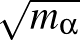with 1≤α≤3N for N atoms. Here we follow the notation in Energy Landscapes' around p. 141: the matrix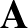diagonalises the mass-weighted Hessian h, and the corresponding eigenvectors of h are orthonormal. As shown in equation (2.51) of Energy Landscapes', the relative displacements for Cartesian coordinate Xα are then Aαγ/, as printed in the vector.dump file. Hence, to put kinetic energy of magnitude kγ into motion corresponding to normal mode γ requires Cartesian velocities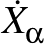= ±Aαγ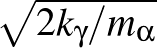, choosing either the plus or minus sign consistently for a given mode γ. • CASTEPC job system: tells the program to use the new CASTEP to calculate energies and forces with input files based on the system name system. In this case the system is assumed to be a cluster, so that both translational and rotational degrees of freedom correspond to zero Hessian eigenvalues at a stationary point. job is a string in quotes that specifies the system call required to run the CASTEP executable. If MASS and DUMPVECTOR ALLVECTORS are set in odata then the normal mode frequencies will be printed in wavenumbers, and the normal mode eigenvector components will be transformed to the Cartesian basis from the mass-weighted Cartesian basis. It is necessary to specify the atoms in order of increasing atomic number, since otherwise they are reordered by CASTEP. There is now a check for this in OPTIM. The PARALLEL keyword is no longer needed, since the number of processors can be specified in job if necessary. Examples for mek-quake, darwin and zippo: CASTEPC 'mpirun /home/wales/bin/castep' 'qge' CASTEPC 'mpirun -np 64 -machinefile machine.file /home/dw34/bin/castep.mpi /home/dw34/bin/castep.mpi' 'qge' CASTEPC 'srun -p sca -n 36 /home/wales/bin/castep' 'qge' For a CASTEP or ONETEP normal mode analysis set STEPS 0, MASS, ENDHESS, and ENDNUMHESS in odata. The Hessian eigenvalues and normal mode frequencies are printed to standard output automatically. Check pertable.f first to make sure that the atomic mass is known for all the elements in your system. The frequency conversion assumes that the units of energy and distance are electron volts and Å. If DUMPVECTOR ALLVECTORS is set in odata then all the normal mode eigenvector components will be transformed to the Cartesian basis from the mass-weighted Cartesian basis. The components printed in the vector.dump file for normal mode γ are then Aαγ/with 1≤α≤3N for N atoms. Here we follow the notation in Energy Landscapes' around p. 141: the matrixdiagonalises the mass-weighted Hessian h, and the corresponding eigenvectors of h are orthonormal. As shown in equation (2.51) of Energy Landscapes', the relative displacements for Cartesian coordinate Xα are then Aαγ/, as printed in the vector.dump file. Hence, to put kinetic energy of magnitude kγ into motion corresponding to normal mode γ requires Cartesian velocities= ±Aαγ, choosing either the plus or minus sign consistently for a given mode γ. • CHARMM: specifies that the CHARMM potential is used. An auxiliary file input.crd is required. CHARMM must be the last OPTIM directive in the odata file. The remaining content of odata consists of CHARMM keywords and setup information. • CHARMMDFTB: specifies that the CHARMM SCC-DFTB potential is to be used, and disables updates of the nonbonded list. This assumes you are using a fully QM system. If you are using a QM/MM setup, you should not use this keyword! Note that SCC-DFTB can only be used with CHARMM35. • CHARMMNOTUPDATE: When using a very large cutoff, turn off nonbond list updating entirely. • CHARMMTYPE topfile paramfile: topfile and paramfile are the common CHARMM top and param files , e.g., toph19_eef1_perm.inp' and param19_eef1_perm.inp'. • CHDEBUG EDEBUG: produces more CHARMM related printing. Turned off by default. If EDEBUG is given as argument, after each CHARMM energy call the energy is decomposed and the components printed. Produces a lot of output! • CHECKD n: for n = 1 (the default) prints numerical and analytical gradients for the starting coordinates, then exists. For n = 2, do the same for second derivatives. For n = 0 simply print the energy and exit. • CHECKINDEX nevs ceig nevl: instructs the program to check the Hessian index after a BFGS minimisation or BFGS hybrid transition state search. This keyword also works with NOHESS. The parameters are the same as for BFGSTS below, and need not be set if they have already been specified by that keyword. • CHECKCHIRALITY: checks that no inversion of a chiral centre happens. Residues can be L or D, but should remain in the same enantiomeric state during the simulation. CHECKCHIRALITY is always turned on for CHARMM, AMBER9 and NAB, i.e. this line is not needed in the odata file. • CHECKCONT: if CHECKINDEX is specified then CHECKCONT instructs the program to take a pushoff and continue if convergence to a stationary point with the wrong Hessian index is detected. • CHECKGRAD diff: calculate numerical two-sided first derivatives using displacement diff and compare with analytical values. Then stop. • CHECKHESS diff: calculate numerical two-sided second derivatives using analytical first derivatives and displacement diff for comparison with analytical values. Then stop. • CHECKNEGATIVE: if set then in bfgsts we backtrack and reduce the maximum step size if the smallest non-zero eigenvalue is positive. If the product of the scale factor with the maximum eigenvector-following step size and with the maximum LBFGS tangent space minimisation step size falls below the minimum value of the maximum step size set by MINMAX then the transition state search is aborted. • CHECKOVERLAP x: if set then in bfgsts we backtrack and reduce the maximum step size if the magnitude of the dot product between the eigenvector corresponding to the uphill direction for the current and previous steps falls below x (default 0.1). If the product of the scale factor with the maximum eigenvector-following step size and with the maximum LBFGS tangent space minimisation step size falls below the minimum value of the maximum step size set by MINMAX then the transition state search is aborted. • CHECKREP n x specifies the interval for updating the neighbour list for repulsive terms when INTCONSTRAINT is used. n is the interval for checking the neighbour list in terms of minimisation cycles for the constraint potential, default 10, and x is the multiple of the repulsive cutoff distance for which sites are included in the list, default 2.0. See also CONINT, CONCUTABS, INTFREEZE, MAXCON, INTIMAGE, and INTCONSTRAINT. • CHINTERPOLATE bint sint DNEB: specifies the interpolation between endpoints for CHARMM. Following specifications are possible: CHINTERPOLATE BC SC does the interpolation for BHINTERP in Cartesians for the backbone and sidechains. That's the default for BHINTERP, which in this case also works without that line. CHINTERPOLATE BC SI does the interpolation for BHINTERP in Cartesians for the backbone and in Internals for the sidechains. CHINTERPOLATE BI SI does the interpolation for BHINTERP in Internals for the backbone and sidechains. CHINTERPOLATE BC SC DNEB does the interpolation for DNEB in Cartesians for the backbone and sidechains. That's the default for DNEB, which in this case also works without that line. CHINTERPOLATE BC SI DNEB does the interpolation for DNEB in Cartesians for the backbone and in Internals for the sidechains. CHINTERPOLATE BI SI DNEB does the interpolation for DNEB in Internals for the backbone and sidechains. The Internals are the primitive CHARMM internal coordinates. If you would rather like to use the natural internals, you have to use the INTINTERP keyword • CISTRANS: allows cis-trans isomerisations for CHARMM, AMBER9 and NAB. • CLOSESTALIGNMENT: specifies a job in which the structure supplied in the finish file is optimally aligned with the first set of coordinates given as input, the minimized distance is printed, and the aligned finish coordinates are dumped in an appropriate format (aligned.crd for CHARMM, aligned.rst for AMBER/NAB and aligned for everything else). • CLASSICALRATES temp1 temp2 tempstep : Don't mix wit CALCRATES and READPATH, which are obsolete. • CLSTRING: Use the climbing string method for saddle point search. (See Ren and Vanden-Eijnden, J. Chem. Phy., 138, 134105, 2013.) Should be used in conjunction with the GROWSTRING keyword to set parameters. Set EVOLVESTRING keyword to be true implicitly. The whole process will converge when the RMS of the last image meets the converge criterion. • COLDFUSION thresh: if the energy falls below threshold thresh then cold fusion is assumed to have occurred and geometry optimisation stops. • COMMENT or NOTE: the rest of the line is ignored. • CONCUTABS x specifies the cutoff for the constraint potential when INTCONSTRAINT is used. Distances that deviate from the reference value by less than x, default 0.15, are not penalised by the constraint potential. See also CONINT, CHECKREP, INTFREEZE, MAXCON, INTIMAGE, and INTCONSTRAINT. • CONNECT n: find a min-sad-min- ... -min sequence connecting the initial minimum in odata (or auxiliary files for CHARMM, UNRES, etc.) to the final minimum in finish, giving up if more than n transition states are needed (default n=100). CONNECT generally needs to be augmented by other keywords to specify how the transition state geometries should be guessed (NEB, NEWNEB, FIXD or GUESSTS), how the transition state searches should be performed (SEARCH 2 or BFGSTS, with or without NOHESS, NOIT etc.), and how that pathways should be calculated (SEARCH 0, 6 or 7, BFGSMIN, RKMIN or BSMIN). A summary file will be produced if DUMPPATH or DUMPALLPATHS is specified. An xyz file for the overall path will be printed to path.xyz and the energy as a function of the path length is printed to EofS. The corresponding xyz and energy files for the individual steps in the path are numbered path.n.xyz and EofS.n for transition state n. This keyword should NOT be used unless there's a really good reason - use NEWCONNECT instead. • CONSEC nstart nend nstart nend etc.: construct linearly interpolated, internal coordinate transition state guesses and apply the stopping criterion in a CONNECT run over a chosen segment of protein specified by pairs of residue numbers (nstart,nend). Up to ten distinct sections can be chosen. • CONINT specifies that terms for internal minimum distances should be included in the energy and gradient for the auxiliary interpolation potential when INTCONSTRAINT is specified. See also CONCUTABS, CHECKREP, INTFREEZE, MAXCON, INTIMAGE, and INTCONSTRAINT. • CONVERGE x y NOINDEX: change the default convergence conditions for eigenvector-following and second order steepest-descent calculations described in §5. If one number is supplied then convergence depends only upon the maximum unscaled step falling below the specified value (and the right number of negative Hessian eigenvalues). If two numbers are supplied the second is the required threshold for the RMS force which must also be satisfied. If the string NOINDEX is present then the Hessian index isn't checked. • COPYRIGHT: prints copyright information. • CPMD system: tells the program to use CPMD to calculate energies and forces with input file system; bulk boundary conditions. • CPMDC system: tells the program to use CPMD to calculate energies and forces with input file system; cluster boundary conditions. • CPPNEB: specifies that the C++ implementation of the DNEB algorithm should be used. For this keyword to work, you must first download the C++ source code into OPTIM/cppneb/external (running the script OPTIM/source/setup.sh does this automatically). You must also compile OPTIM with the WITH_CPPNEB option set, and then use the executable OPTIM_CPPNEB. NOTE: the C++ code expects that the variable NEBK will be set to the value of half the actual spring constant. If you are translating between the Fortran and C++ implementations of DNEB, you will need to adjust your NEBK accordingly. • CUBIC: if the three box lengths are equal to start with in a PV run, and the keyword CUBIC is included, then a cubic box is maintained. • CUBSPL: For the growing string or evolving string double-ended transition state search methods, use a cubic spline interpolation between the image points. • CUDA potential: specifies a GPU run. Setting potential to 'A' specifies the AMBER12 potential. See the group wiki for further information. • CUTOFF rcut: Specify that the potential should go be zero for atom separations larger than rcut. This is not suppored for all potentials. (For some potentials rcut is passed using the PARAMS keyword.) • D5H x: add a decahedral field of strength x to the potential. • DB epsbb epsab sigmabb sigmaab muD E: calls a finite system of dipolar Lennard-Jones dumbbells , where the electric field of strength E can be optionally present. The field, if present, is along the space-fixed z-direction. εaa and σaa are both set to unity by default. μD is the dipole moment; ε's and σ's correspond to the Lennard-Jones parameters. • DCHECK ON/OFF: turns on/off warning messages when atoms approach to within 0.5 distance units. Default is ON. • DEBUG: causes unscaled steps, gradients, Hessian eigenvalues and summary information to be printed every cycle for SEARCH 0–7 runs. Turned off by default. Also produces much more printing for individual steps in CONNECT runs. • DESMAXAVGE E: specifies a maximum average energy, such that double-ended search methods will not stop until the average energy of the images is below E. • DESMAXEJUMP E: specifies a maximum energy jump, such that a particular image step in a double-ended search method is skipped if the starting energy of that image is below 0 and the energy increases by more than E. Particularly useful when the DESMINT keyword is set. Recommended value 1.0×104. • DESMDEBUG: Produces extra printing for the double-ended transition state search method runs (DNEB, GS or ES). • DF1: specifies a binary 2D potential. The first N/2 atoms have unit radius and the rest have radius 1.4, with a cutoff for each pair type at the average radius. The keyword 2D must also be specified, along with a PARAMS line to specify two box-lengths. Initial work uses box lengths of 3.31437171 for a number density of 0.9. • DFTB: specifies that the tight-binding code of Walsh and Wales is used. • DFTBP: specifies that the DFTBP (SCC-DFTB+) code is used. The required files are dftb_in.hsd and the relevant Slater-Koster parameter files (*.skf) for each atomic pair in the system under study. These SK files can be downloaded from: https://dftb.org/parameters/download/. The geometry should always be specified in the dftb_in.hsd file and can also be read from a start file if needed. The optimisation is conducted in fractional coordinates for geometry specifications F' and S'. Hence, when S' input is detected the coordinates in the dftb_in.hsd file are converted to fractional. In this case, the same conversion is performed for coordinates read from a start file and for finish if relevant. To build DFTBPOPTIM either use Intel and an mkl library, or GCC. Other modules may interfere. This combination works on nest for Intel: ifort/64/2020/4/304, mkl/64/2022/0/0, cmake/3.23.2. For ifort the icc compiler needs to be specified as well: FC=ifort CC=icc CXX=icc cmake ∼/softwarewales/GMIN/source/ -DWITH_DFTBP=yes If using GCC, a system BLAS needs to be used. Depending on the machine, CMake may be able to find one, or you may have to specify one yourself. This combination of modules works on nest: gcc/12.2.0, cmake/3.23.2, with a configure line of cmake ∼/softwarewales/GMIN/source/ -DWITH_DFTBP=yes -DWITH_MYBLAS=no -DBLAS_LIBRARIES=/usr/lib64/libopenblas.so.0 To build DFTBP standalone in the DFTBP directory use FC=ifort CC=icc cmake -DCMAKE_INSTALL_PREFIX=HOME/softwarewales/DFTBP/_build -B _build .

then

cmake --build $HOME/softwarewales/DFTBP/_build -- -j then cmake --install$HOME/softwarewales/DFTBP/_build

• DISTCON file: specifies a general atom-atom harmonic distance constraint potential, which is added to the underlying potential. file is a file containing a list of atom pairs, the reference distance, and the force constant used in the harmonic term that penalises deviation from the reference separation. For example
367 393 2.27 1.01D3
372 385 2.65 1.02D3

• DGUESS dguess1 dguess2 dguess3 dguess4: initial guess for diagonal elements of the inverse Hessian, used whenever the BFGS optimiser is reset. dguess1 is used in geometry optimisations, dguess2 is used for minimisation in eigenvalue calculations, dguess3 is used for DNEB optimisation steps, and dguess4 is used for string method optimisation steps. The default values are dguess1=dguess2=0.1, dguess3=dguess4=0.001

• DIJKSTRA [EXP/n/INDEX/DIHEDRAL n] [INTERP x EXP/n]: specifies that the missing connection Dijkstra-based algorithm should be used to choose minima for connection in cycles of NEWCONNECT. The edge weights are based on the smallest distance between minima exponentiated (for EXP) or raised to the integer power n; if INDEX is specified instead then the metric becomes the modulus of the difference between the positions of the minima in the order they were found. Alternatively, the additional keyword INTERP specifies an edge weight based on the highest energy difference found by a linear interpolation between the minima at regular spacings of x. If n is also specied then the metric is raised to the power n. The final EXP or integer n specifies whether this highest energy is exponentiated or raised to the power n to obtain the edge weight. DIHEDRAL specifies an alternative metric for AMBER12 or AMBER20 based on dihedral angles. Here we sum the sine of the difference in dihedral angles for two structures divided by two, squared: sin2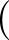θ1 - θ2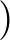/2.

• DNEBEFRAC x: allows more DNEB steps if the current DNEB energy value is greater than DNEBFRAC times the lowest value found so far. Can be useful to prevent premature DNEB exit if we happen to be going through a phase with bad geometries. A maximum of ten times the maximum iterations parameter is permitted.

• DNEBPATH: creates path.info files based on the DNEB images without any further refinement, so long as the CONVERGE and BFGSMIN thresholds are set to huge values so that the images satisfy the RMS gradeint convergence condition. Local maxima in the set of images are taken as transition states, and local minima on either side are assigned as the connected minima'. In DPS runs based on this approach we may have DNEB pathways with endpoints that are local maxima. In this case the image is added as both the fake transition state and the connected minimum.

• DOINVERSION: forces inversion check of structures for distance metric in minpermdist, even for biomolecules.

• DOUBLE: adds a double-well potential as per J. Chem. Phys., 110, 6617, 1999. The energy and derivatives add to the potential specified by the atom type. Only atom type LS' (not binary) has the 1,2 interaction removed as well. At low density some atoms may not interact with any others and problems result due to additional zero eigenvalues.

• DQAGKEY n: n (integer between 1 and 6) indicates the number of local integration points used for calculating cubic spline arc lengths in the string methods. Default value is 6, which corresponds to 30-61 points.

• DRAG: obsolete method that transforms one end point into another by progressively increasing a spring potential that attracts the system to the final geometry.

• DUMPALLPATHS: prints a summary of every min-sad-min path found during a CONNECT or NEWCONNECT run to file path.info. For each stationary point the energy, point group order and symbol, Hessian eigenvalues and coordinates are given. Hessian eigenvalues are computed for all stationary points. This min-sad-min format corresponds to the TRIPLES keyword in the PATHSAMPLE program.

• DUMPBESTPATH: after the last cycle in NEWCONNECT another Dijkstra analysis is run, the best path according to this analysis is then written to path.info. Useful to prevent a fast increase in the database size in PATHSAMPLE.

• DUMPFRQS: if the frequency of a stationary point is calculated, it is written to a file frqs.dump. The keyword is mainly meant for the GETMINFRQS and GETTSFRQS options in PATHSAMPLE. It requires that ENDHESS or ENDNUMHESS are used.

• DUMPDATA: creates a file sp.data.info in PATHSAMPLE min.data or ts.data format for the minimum or transition state found following a minimisation or ts search. The connectivity information will not be available for a transition state; it is better to create path.info file and use PATHSAMPLE keyword ADDPATH if a full transition state entry is required. DUMPDATA was originally intended for setting up a discrete path sampling initial path run by creating entries for the two endpoint minima. Now it can also be used with the CHECKTS/GETTSFRQS procedure in PATHSAMPLE.

• DUMPDATA_MACHINE ndof: to be used instead of DUMPDATA when lots of data will be produced (e.g. using MULTIJOB). The normal output from DUMPDATA is split into two files. The energy, frequency log product, point group order and moments of inertia of each minimum found are written to min.data.info as normal (formatted output). The coordinates of the minima are written to an unformatted file min.data.info, which readable only by the machine and therefore is quicker to read/write and takes up less disk space. Each minimum is written as a single record of length ndof, which is the number of coordinates (degrees of freedom) in the system.

• DUMPEVALS: dumps the hessian eigenvalues in file evals.dump, preceded by the energy, all on one line (hence it has Nvars + 1 columns with N). With MULTIJOB you can obtain results for a set of configurations in one job.

• DUMPINTEOS n: creates the file EofS.int for QCI runs. n is the dump frequency.

• DUMPINTXYZ n: creates the file int.xyz for DNEB and QCI runs. n is the dump frequency.

• DUMPMODE n1 n2 etc: must be used with and below DUMPVECTOR ALLVECTORS MWVECTORS. Produces PDB files mode.n1.pdb, mode.n2.pdb etc containing information on the relative per atom and per residue displacement caused by that mode. This information can be visualised in VMD by colouring with the Beta (per residue) or Occupancy (per atom) options.

• DUMPNEBEOS n: creates the file EofS.neb for DNEB runs. This file is also created if DEBUG is set; the default is false. n is the dump frequency.

• DUMPNEBPTS: saves points for DNEB every iteration.

• DUMPNEBXYZ n: creates the file neb.xyz for DNEB runs. This file is also created if DEBUG is set; the default is false. n is the dump frequency.

• DUMPPATH: prints a summary of the min-sad-min- ... -min path produced by CONNECT or NEWCONNECT to file path.info. For each stationary point the energy, point group order and symbol, Hessian eigenvalues and coordinates are given. Hessian eigenvalues are computed for all stationary points. The path.info format for DUMPPATH is now the same as for DUMPALLPATHS, i.e. min-sad-min then min-sad-min, etc.

• DUMPSP: specifies that information for all the stationary points located should be dumped in PATHSAMPLE min.data/ts.data format.

• DUMPSTRUCTURES: specifies that final structures should be dumped for the Amber 9 runs into pdb, Amber restart and xyz formats.

• DUMPVECTOR [ALLSTEPS] [ALLVECTORS [MWVECTORS]]: if present the Hessian eigenvectors will be written to file vector.dump after each OPTIMisation step, preceded by the corresponding eigenvalue for each one. The eigenvectors corresponding to zero eigenvalues are excluded. For hybrid eigenvector-following/BFGS transition state searches only the results corresponding to the smallest non-zero eigenvalue are dumped. The default is to dump only the vector corresponding to the smallest non-zero eigenvalue for the last step. If ALLSTEPS is present then vectors are dumped at every step. If ALLVECTORS is present then all the vectors corresponding to non-zero eigenvalues are dumped, rather than just one of them. If MWVECTORS is also specified, the vectors dumped are the normal modes, using appropriate mass weighting and the vector.dump file will contain the vibrational frequencies (in wavenumbers) rather than the eigenvalues. Two additional output PDB files are also be produced. totalmodes.pdb contains per atom and per residue displacement information resulting from a vector sum of ALL normal modes of non-zero frequency. weightedmodes.pdb contains similar information for a Boltzmann weighted (by frequency) vector sum of all normal modes. See DUMPMODE for information on how to visualise this data. Note that the Boltmann weighting is done with kT=207.11 cm-1 (corresponding to room temperature) unless otherwise specified by the KTWN keyword. A third output file is also produced if KTWN is specified. ALLSTEPS and ALLVECTORS can be present in either order.

• EDIFFTOL x: specifies the energy difference used to identify permutational isomers in CONNECT and NEWCONNECT runs. Default is x= 10-9.

• EFIELD x: specifies that an electric field along the z axis should be included with magnitude x V/Å. For use with TIPnP potentials.

• EFSTEPS n: prints the unscaled steps in the Hessian eigenvector basis every n steps—turned off by default.

• ENDHESS n: calculate analytical Hessian and normal mode frequencies at end of run. To obtain frequencies for UNRES, CASTEP and ONETEP you must specify both ENDHESS and ENDNUMHESS. For an eigenvalue analysis, with no geometry optimisation, specify STEPS 0. To have the Hessian eigenvalues printed to standard output you must specify the DEBUG keyword, except for AMH, CASTEP and ONETEP, where they are printed automatically. n specifies the number of Hessian eigenvalues to be calculated (starting from the lowest), which defaults to all.

• ENDNUMHESS: calculate numerical Hessian and normal mode frequencies at end of run. Required if DUMPPATH or ENDHESS is specified for an UNRES run, in which case it's an internal coordinate Hessian. Also required for a CASTEP or ONETEP normal mode analysis, which also requires STEPS 0 and ENDHESS to be set. To have the Hessian eigenvalues printed to standard output you must specify the DEBUG keyword, except for AMH and CASTEP, where they are printed automatically.

• ENDNUMHESS2 delta: calculate numerical Hessian and normal mode frequencies at end of run. This keyword is different from ENDNUMHESS in that a higher order finite difference is used (Richardson extrapolation), which takes twice as long, but should be more accurate. The optional parameter delta is the finite difference displacement, default value 10-6.

• EIGENONLY ceig cover: instruct the program to perform a search that only estimate the lowest eigenvalue and the corresponding eigenvector. ceig is a double precision parameter that sets the convergence criterion for the calculation of the smallest non-zero Hessian eigenvalue. If NOHESS is set ceig is compared to the RMS force' corresponding to the Rayleigh-Ritz expectation value that is minimised to get the smallest eigenvalue. If the Hessian is used via iteration or NOIT then ceig is compared to the percentage change in the eigenvalue between successive steps. The default value is 1.0, which is more appropriate to the latter case. Smaller values are probably necessary in BFGS/BFGS calculations. cover is a double precision parameter that sets the convergence criterion. The search coverges when the magnitude of the dot product of the estimated mode with the target mode is greater than "cover". The target mode is read from file "target.vector", the first line of which supposes to be the eigenvalue but not read actually. the following lines are read as the target mode. "cover" doesn't have a default value. It works only if it is seted and the parameter "ceig" is then out of function.

• ERROREXIT: indicates that the program should halt on error.

• EXTRAPERM: for use with PERMDIST when allowing the full permutation group is too permissive. Allows the custom specification of what permutations to allow. For example, when dealing with rigid bodies, the body might have a rotation. The permutations are listed in the file extra_perm.allow, which must be present in the working directory. The first line must specify the number of groups in the file. This is followed by the specification of the groups, each of which has the following format:

<Number of permutations in the group><Number of atoms in the group>

<Identity permutation>

<Other permutation>

.

.

.

Each group should probably be a full symmetry group in the mathematical sense, but this is not checked. For example, if there were two rigid bodies that had the form of pentagonal bipyramids, with an allowed five fold rotation axis interchanging the radial atoms, that were 3-7 in each body, extra_perm.allow might be:

2

5 5

3 4 5 6 7

4 5 6 7 3

5 6 7 3 4

6 7 3 4 5

7 3 4 5 6

5 5

10 11 12 13 14

11 12 13 14 10

12 13 14 10 11

13 14 10 11 12

14 10 11 12 13

• EVCUT x: sets a cutoff value for eigenvalues. Eigenvalues of magnitude less than x are treated as zeros and steps along such directions are omitted.

• EVOLVESTRING: use the evolving string method based on the zero-temperature string method of E et al  for double-ended transition state searches. Should be used in conjunction with the GROWSTRING keyword to set parameters.

• EXTRASTEPS x: obsolete; extra minimisation steps are allowed for CHARMM runs proportional to the separation between two minima multiplied by x.

• FILTH n: specifies an integer variable, n, to distinguish output files in parallel landscape jobs. This keyword will be retired shortly in favour of the new command line specification of file name extensions.

• FIXAFTER n: specifies that FIXIMAGE should be set permanently after step n. This effectively freezes the interacting images in different supercells for calculations with periodic boundary conditions.

• FIXATMS : For the GS and ES double-ended transition state search methods, avoid overall translation and rotation by fixing all three coordinates of one atom, confining another atom to a line, and a third atom to a plane for all steps.

• FIXD t12fac dthresh: transition state search using hard sphere moves. A random velocity vector is assigned and the first hard sphere collision is located. If t12fac≤1 then the system is moved through a fraction t12fac of this first collision time; the default value is 1. If t12fac> 1 then the two atoms are moved half way between the entrance and exit of their collision, but their separation is rescaled to unity. See also RANSEED. Used in CONNECT procedure only if FIXD is set and the two minima to be connected are closer than dthresh.

• FRACTIONAL: specifies fractional coordinates to be used in box length optimisation for constant pressure runs using PV.

• FREEZE n1 n2 etc.: specifies that atoms n1, n2, etc. should be frozen. Only works for LBFGS minimisation and BFGSTS if 3 or more non-linear atoms are frozen. Frozen atoms may also be specified in a file frozen. The first line of the file should contain the number of frozen atoms. Subsequent lines contain the atom indices to be frozen.

• FREEZERES n1 n2 etc.: specifies that residues n1, n2, etc. should be frozen.

• FREEZEGROUP centreatom radius mode: specifies that all atoms outside (mode = GT, the default) or within (mode = LT) a sphere of radius radius centred on atom centreatom should be frozen. Also produces a file frozen.dat containing the corresponding single atom FREEZE commands.

• FREEZENODES tol: For the double-ended transition state search methods (NEB, DNEB, GS, ES), at each step freeze those nodes for which the RMS perpendicular force, as a fraction of the RMS force over all the images, is less than tol. over all the images.

• FREEZERANGE n1 n2 : freezes all atoms in the range of atom numbers n1 to n2.

• FRQCONV x: Specify a conversion factor x to be applied to Hessian eigenvalues and frequencies before they are printed, either in min.data.info files as the log product of Hessian eigenvalues (log product of square angular frequencies) or in path.info files as the actual frequencies. The default factor x is 1, which is appropriate for systems where all atoms have the same mass. In this case, frequencies are given in internal units of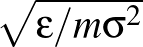where ε is the energy unit, m the mass unit and σ the length unit for the system in question. For more complex systems (particularly external potentials such as AMBER) it may be more convenient to convert to SI units before printing out. The most common potentials have default values of x hard-coded into keywords.f, but specifying this keyword will override all defaults if you wish to work in a non-SI unit system. For more detail on how this keyword works, check the comments in keywords.f.

• GAMESS-UK system exec: potential will be generated by GAMESS-UK. system is a string to identify the system and exec is the name of an executable that will generate a GAMESS-US input deck from a points file. If exec is omitted its name is assumed to be editit.system.

• GAMESS-US system exec: potential will be generated by GAMESS-US. system is a string to identify the system and exec is the name of an executable that will generate a GAMESS-US input deck from a points file. If exec is omitted its name is assumed to be editit.system.

• GAUSSIAN: tells the program to read derivative information in Gaussian92 format. This should not be used; it has been superceded by GAUSSIAN09 and friends.

• GAUSSIAN03 charge multi: potential will be generated by Gaussian03. charge is the total charge of the system. multi: is the spin multiplicity of the system. Note that Gaussian outputs energy derivatives in atomic units. OPTIM expects coordinates in Angstrom, and converts the units of the Gaussian derivatives. An example can be found in: http://www-wales.ch.cam.ac.uk/examples/OPTIM/

• GAUSSIAN09 charge multi: potential will be generated by Gaussian09. charge is the total charge of the system. multi: is the spin multiplicity of the system. Note that Gaussian outputs energy derivatives in atomic units. OPTIM expects coordinates in Angstrom, and converts the units of the Gaussian derivatives. An example can be found in: http://www-wales.ch.cam.ac.uk/examples/OPTIM/

• GAUSSIAN16 charge multi: potential will be generated by Gaussian16. charge is the total charge of the system. multi: is the spin multiplicity of the system. Note that Gaussian outputs energy derivatives in atomic units. OPTIM expects coordinates in Angstrom, and converts the units of the Gaussian derivatives. An example can be found in: http://www-wales.ch.cam.ac.uk/examples/OPTIM/

• GEOMDIFFTOL x: specifies the distance criterion used to identify identical isomers in CONNECT and NEWCONNECT runs. Default is x= 10-1.

• GLJ n1 n2 n3 etc.: specifies general Lennard-Jones potential for any number of differ LJ species. The ε and σ parameters for all the different species should be specified as the lower diagonal blocks of the corresponding matrices on the following lines. For example, to specify a 13-atom cluster with three different species: GLJ 6 4 3 1.0 1.1 1.2 1.2 1.3 1.4 1.0 1.5 1.6 1.7 1.8 1.9

• GOT: Paul Whitford's Gō model potential.

• GRADIENTS n: causes the gradients along the Hessian eigenvectors to be printed every n cycles. Gradient printing is turned off by default.

• GRADSQ gsthresh nspecial nallow specifies optimisation of the modulus gradient with nallow second order steps at intervals of nspecial if the RMS force lies below gsthresh.

• GRADTYPE type specifies the gradient formulation to use with the NEWNEB keyword. The default is dneb'; other allowed values are jnew' (new implementation of neb by Jonsson), spr' (full springs), jold' (springs MEP-parallel component only, the original formulation), nper' (springs MEP-parallel component (original formulation) + n% of perpendicular component), nper2' (springs MEP-parallel component (original formulation) + n% of perpendicular component, possibly optimised) dneb2' (same as dneb', except spring gradient usage is more consistent). dihen' (distance between images calculated in dihedral angle space; for use with CHARMM and UNRES), dnebu' (DNEB for unres), dneb3' (uses deviation from the average separation in the spring term instead of absolute distance),

• GREATCIRCLE gcmxstp gcmxstp gcsteps gcconv: obsolete; interpolation between two end points using a great circle in 3N-dimensional space.

• GROWSTRING nimage iterd reparamtol growtol convtol mxstp: specifies that double-ended transition state searches will be done using either the evolving string method (based on that described by E et al) or the growing string method (based on that described by Peters et al). The default is growing strings; if the separate keyword EVOLVESTRING is also specified, the evolving string method will be used instead. nimage and iterd specify the number of images and the maximal iterations per image to be used in the first connect run; for all subsequent connections these parameters are determined from the NEWCONNECT line. In the growing string case, the iteration density affects only the maximum iterations allowed after the string is fully populated with images. The string is reparametrised and the images redistributed whenever the ratio of actual inter-image distance to the desired distance falls below reparamtol for at least one segment. For growing strings, a new image is added to one side of the string whenever the RMS perpendicular force on one of the frontier images falls below growtol. The string is deemed to have converged if the RMS perpendicular force over all the images falls below convtol. mxstp refers to the maximum allowed step for each image during the optimisation. The default is a linear interpolation between image points; use the CUBSPL keyword to switch this to a cubic spline interpolation.

• GSMAXTOTITD itd: Set the maximum total iteration density for the growing string method. This specifies the maximum evolution iterations allowed per total image number, including the iterations while the string is still growing. If itd is less than 0, then this parameter is turned off and there is no limit on the total iterations (this is the default).

• GUESSPATH gsteps maxgcycles gthreshold maxinte: obsolete; try to guess an interpolated path for sequential coordinate changes. gsteps is the number of step to be tried for each sequential coordinate. maxgcycles is the number of sweeps through pairwise exchanges gthreshold is the coordinate change above which sequential changes are considered. maxinte is the convergence criterion for the maximum allowed interpolated energy.

• GUESSTS guessthresh: use dihedral twisting in place of NEB for transition state guesses with CONNECT for CHARMM and UNRES.

• HESSDUMP: dump the Hessians for local minima and transition states in files minhess.plus, minhess.minus, and tshess. The Hessian is printed before each diagonalisation step that is used to produce entries in path.info files in subroutines path and ncutils. If more than one minimum-transition state-triple appears in the path.info file then the corresponding Hessians are simply concatenated in the hess files. Only works if DUMPPATH or DUMPALLPATHS are set.

• HESSGRAD: For the growing string or evolving string double-ended transition state search method, use the method described in the appendix of Peters et al to calculate the Newton-Raphson search direction. Namely, the Hessian is approximated based on changes in the gradient, and the tangential component of - Hf is projected out. By default, the Hessian used is actually an approximation to the derivative matrix of f rather than the gradient.

• HIGHESTIMAGE: obsolete; only use the highest non-endpoint image in a double-ended MECCANO run.

• HUPDATE nstart ninterval phi: uses a Hessian update procedure instead of calculating the analytic second derivatives at every step. nstart is the first step at which analytic derivatives will be calculated; by default nstart=0, the Hessian is initialised to the identity and no analytic second derivatives are ever calculated. ninterval is the interval at which analytic Hessians are calculated; the default is 0, which means that no additional analytic Hessians are ever found. A transition state search starting from a minimum seems unlikely to work unless we calculate analytic Hessians at regular intervals including the first step. Update applied is phi times Powell plus (1-phi) times Murtagh-Sargent. (See Bofill and Comajuan, J. Comp. Chem., 11, 1326, 1995.)

• HYBRIDMIN nevs ncgmax1 ncgmax2 ceig mxstp nsteps evmax method nevl: instructs the program to calculate pathways using hybrid minimisation. nevs is an integer that defines the largest number of iterations allowed in the calculation of the smallest Hessian eigenvalue; default 500. ncgmax1 is an integer that defines the largest number of BFGS steps allowed in the subspace minimisation before the eigenvalue is deemed to have converged; default 10. ncgmax2 is an integer that defines the largest number of BFGS steps allowed in the subspace minimisation after the eigenvalue is deemed to have converged; default 100. This parameter is ignored if NOIT is set. The eigenvalue is deemed to be converged if the modulus of the overlap between the corresponding eigenvector and that saved from the previous step exceeds 0.999 and we have the right number of negative eigenvalues. ceig is a double precision parameter that sets the convergence criterion for the calculation of the smallest non-zero Hessian eigenvalue. If NOHESS is set ceig is compared to the RMS force' corresponding to the Rayleigh-Ritz expectation value that is minimised to get the smallest eigenvalue. If the Hessian is used via iteration or NOIT then ceig is compared to the percentage change in the eigenvalue between successive steps. The default value is 1.0, which is more appropriate to the latter case. Smaller values are probably necessary in BFGS/BFGS calculations. mxstep is the maximum initial step size allowed for the step along Hessian eigenvectors; it is adjusted dynamically using a trust radius scheme (see TRAD). nsteps is the maximum number of steps before returning and switching to LBFGS minimisation. The routine will also exit and switch to LBFGS if the smallest non-zero Hessian eigenvalue exceeds evmax. method can be either EF' or PM', specifying eigenvector-following or Page-McIver steps. The number of non-zero Hessian eigenvalues and corresponding eigenvectors determined is one by default, but any value can be specified using USEEV.

nevl is an integer that defines the largest number of iterations allowed in the calculation of the largest Hessian eigenvalue; default 100. Not needed if NOHESS of NOIT is set.

• IH x: add an icosahedral field of strength x to the potential.

• INDEX n: initiates a search for a saddle of index n if SEARCH 2 is specified. See also KEEPINDEX. Also works with BFGSTS up to a maximum of index 50, but NOIT must be set and a Hessian is needed.

• INE_NEW sttsrmsconv st_tsstep lan_dist lanstep lanconv lanfactor: Using "inexact newton method" to accelerate the convergence of TS search at the end of the climbing string method. sttsrmsconv sets is the convergence criterion for the root-mean-square gradient. st_tsstep sets the maximum number of steps allowed to move. lan_dist sets the small parameter used to approximate the matrix-vector multiplication. lanstep sets the maximum iteration number of Lanczos. lanconv sets the stop criterion of Lanczos process. lanfactor sets a scale parameter to control the step size of step vector.

• INSTANTONOPT nimageinst distortinst deltainst temperature: Instanton optimisation with nimageinst is the number of images used in the optimisation, distortinst the amount of distortion from the classical transistion state to obtain the initial instanton path, and deltainst the offset to calculate the instanton Hessians. Example parameters are: nimageinst=1, distortinst=0.4, deltainst=0.01. The temperature for the tunnelling process is specified only with this keyword.

• INSTANTONRATE nimageinst : Instanton rate calculations. For more details see 14.

• INSTANTONSTARTDUMP temperature: Dumps the initial files for instanton rate calculations. The file input.xyz contains either the transistion state (saddle point) or the reactant state (minimum). For more details see 14.

• INTEPSILON x: specifies the value of the ε parameter for diagonal shifts in the sparse internal coordinate transformation. The default is x = 10-6.

• INTERPBACKTCUT c: the internal coordinate back-transformation convergence cutoff used for interpolations. Default value is 1.0×10-8

• INTERPCHOICE: when used with the INTINTERP keyword, specifies that linear interpolations between endpoints are done with both internal and Cartesian coordinates. The interpolated path with the lower maximum energy is then used to start the double-ended search method.

• INTERPSIMPLE: when used with the INTINTERP keyword, specifies that an internal coordinate interpolation is done with the same number of image points as desired for the double-ended search methods. The resulting image points are converted directly to Cartesians. The Cartesian images will not necessarily be evenly distributed along the path.

• INTINTERP nim: specifies that internal coordinates are to be used for interpolating between endpoints. Automatically uses natural internal coordinates. Does a linear interpolation with nim images, then places the required number of Cartesian image points at approximately equal arclengths along the resulting path. Default value of nim is 100. Turns off the DESMINT keyword.

• INTMIN: specifies that internal coordinates be used in calls to mylbfgs, including minimisation in the tangent subspace for hybrid eigenvector-following. The algorithm used is that of Németh et al. Not recommended.

• INTMINPERM: when aligning endpoint structures, permute the permutable atoms to minimise the distance in individual torsion angles. Use only with INTINTERP or DESMINT.

• INTPARFILE file: Use a parameter file to specify the internal coordinates for amino acids, rather than generating them automatically.

• INVERTP: specifies the inverted potential. The energy and all derivatives are multiplied by -1. This converts searches for the highest index saddles into minimisation. However, it is only likely to work for bounded potentials.

• KEEPINDEX : specifies that INDEX is set equal to the number of negative Hessian eigenvalues at the initial point.

• KTWN x : for use with DUMPVECTOR ALLVECTORS MWVECTORS - sets kT in cm-1 to x. The default value is 207.11 cm-1. If KTWN is specified, an additional output file, thermalmodes.pdb is produced during a normal mode analysis containing per atom and per residue displacement information resulting from a Boltzmann weighted (by frequency) vector sum of all normal modes whose frequencies are less than kT. This information can be visualised as described for DUMPMODE.

• LANCZOS : obsolete; specifies that a Lanczos procedure should be used to find eigenvalues and eigenvectors. Lapack routine DSYEVR is now used throughout instead.

• LAMMPS: invokes the LAMMPS interface, where energies and gradients are obtained from the LAMMPS package. This keyword requires file in.lammps in the working directory to set up the LAMMPS input and the initial coordinates. The LAMMPS interface assumes an MPI compilation. The current build has been tested on sinister and nest for branch DJWlammps, and has hardcoded libraries. It works with modules cmake/3.16.3, gcc/7.5.0, mpi/openmpi/gnu7/4.1.0, and the cmake line is
FC=mpif90 CC=mpicc CXX=mpicxx cmake -DWITH_LAMMPS=1 /softwarewales/GMIN/source/ -DCOMPILER_SWITCH=gfortran -DWITH_MPI=yes

• LJADD: specifies an addressable LJ potential. A data file epsilon is required specifying the well depth parameter ε for all pairs of atoms, one per line. Choosing values according to nearest neighbours in a reference geometry can bias the system towards that configuration.

• LJADD2 n: specifies an addressable LJ potential for multiple copies of an n-particle cluster. The data file epsilon is required with n×n entries, one per line. These well depth parameters are then applied modulo n. For particles with the same address, only the repulsive part of the LJ potential is used.

• LOCALPERMDIST thresh cutoff orbittol: minimise distances between end points prior to DNEB interpolation using local alignment. thresh is used to define the tolerance for a perfect' alignment in routine minpermdist, which is called for subsets of atoms rather than all at the same time for PERMDIST. The threshold used is actually the number of atoms in the permutable group in question times thresh. The routine minpermrb cycles through the permutable groups, calling minpermdist for each one, augmented by any other permutable groups that overlap, as for any groups with additional pair swaps, such as valine and arginine. Atoms that are equidistant from the permutable atoms in the two end points are also added to the set if the distance lies within a cutoff radius cutoff. They are added in increasing distance order, and removed one at a time if the resulting subsets of atoms cannot be aligned perfectly' between the two endpoints. Using cutoffs of 2.5 and 5 Å gives different internal rotations for an arginine group, but with similar barriers. The default values are 0.1 and 5.0 for thresh and cutoff, respectively. orbittol sets the threshold for assigning atoms to the same orbit when analysing the standard orientation in routine myorient; default is 0.001. Requires the auxiliary file perm.allow to specify permutable atoms, otherwise all atoms are assumed to be permutable. The absence of a perm.allow file is considered a mistake for CHARMM runs. See also LPERMDIST, which effectively replaces LOCALPERMDIST.

The first line of the perm.allow file must contain an integer that specifies the number of primary groups of interchangeable atoms. The groups then follow, each one introduced by a line with two integers p and s that specify the number of permutable atoms in the primary group and the number of other sets of permutable atoms associated with the primary set. s may be zero. Each secondary set of permutable atoms has p members. The following line contains the indices of the p permutable atoms in the primary set and then the indices of the atoms in each of the s secondary sets, one set at a time.

Scripts to generate allowed permutations automatically for CHARMM and AMBER are available from the group web site. It is essential to use symmetrised versions of the corresponding force fields! See the PERMDIST keyword for example perm.allow file entries.

• LOWESTFRQ: specifies that the lowest positive eigenvalues associated with the Hessian and mass-weighted Hessian should be calculated and written to min.data.info. These eigenvalues can then be used in combination with DUMMYTS in PATHSAMPLE to estimate the energy and frequency factor associated with dummy transition states. The LOWESTFRQ keyword must appear in odata.bhinterp or odata.bisect for such jobs, along with DUMPDATA and BHINTERP or BISECT.

• LPERMDIST n thresh cut orbittol: provides the preferred local permutational alignment procedure. n is the maximum number of extra atoms to include in running minperm for each permutable group (default 11, a value that may be needed to treat phenylalanine), rather than treating the whole system at the same time, as for PERMDIST. The extra atoms that define the neighbourhood of the permutable group are added one at a time in order of their distance from the centre of coordinates of the group, averaged over the start and finish geometries. If the optimal aligned distance is greater than thresh (default value 0.5) then the atom is not included in the neighbour list. This procedure continues until there are n atoms in the neighbour list, or no more atoms within the cutoff distance cut, default 5.0. The parameter orbittol is the distance tolerance for assigning atoms to orbits in the myorient standard orientation routine, default value 0.06. This keyword requires the auxiliary file perm.allow to specify permutable atoms, otherwise all atoms are assumed to be permutable. The absence of a perm.allow file is considered a mistake for CHARMM runs.

• MACHINE: specifies that points should be saved in binary (machine) format instead of human-readable xyz. Default false.

• MASS: specifies the use of mass weighting for the steps; the output coordinates are not mass weighted.

• MAXBARRIER x: x specifies the maximum value for the smaller barrier height that is allowed to constitute a connection during the DIJKSTRA connection procedure. See also MAXMAXBARRIER to restrict the larger barrier height.

• MAXBFGS x1 x2 x3 x4: x specifies the maximum allowed step length in LBFGS minimisations, x1 for normal minimisations, x2 for Rayleigh-Ritz ratio minimisation, x3 for putting structures in closest coincidence with ministd, and x4 for NEB minimisations. Default values all 0.2.

• MAXCON n specifies the maximum number of distance constraints for any atom when QCI/INTCONSTRAINT is used. The default value for n is 4.

• MAXERISE x1 x2: x1 is the maximum energy increase above which mylbfgs will reject a proposed step. The default is x= 10-10, but a much larger value may be needed for CASTEP runs. x2 performs the same function for the maximum rise in the Rayleigh-Ritz ratio during minimisation in xmylbfgs; however, it uses the fractional value of the increase relative to the magnitude of the eigenvalue for easier transferability. Hence the dfault value is larger, namley 0.001.

• MAXFAIL n: maximum number of failures to lower the energy allowed in a minimisation before giving up.

• MAXGAP : specifies that in NEWCONNECT runs only one connection attempt is made per cycle, corresponding to the minima separated by the largest gap.

• MAXGROWSTEPS n: For the growing string double-ended connection method, specify a maximum number of steps allowed before another image is added to the growing string. Default is 1000.

• MAXIMFACTOR x: this is the factor used in output.f90 to identify local maxima for hybrid EF ts searches; the default is x = 10. It multiplies EDIFFTOL.

• MAXLENPERIM x: will stop the entire job if the total string length for the growing strings or evolving strings method goes above x times the total number of images. This usually means that something is going wrong with the string. Default is 1000.

• MAXMAX x: specifies the maximum value that the maximum step size is allowed to rise to. The default value is 0.5. MAXMAX is also used as the uphill step size in hybrid eigenvector-following transition state searches if the eigenvalue of the direction being followed is positive.

• MAXMAXBARRIER x: x specifies the maximum value for the larger barrier height that is allowed to constitute a connection during the DIJKSTRA connection procedure. See also MAXBARRIER to restrict the smaller barrier height.

• MAXSTEP x1 x2: specifies the maximum step size for eigenvector-following and steepest-descent calculations, default value 0.2. The precise implementation of this constraint depends upon the step scaling method employed (see §8).

• MAXTSENERGY x: maximum ts energy that is allowed to constitute a connection during the Dijkstra connection procedure. Paths are not calculated for transition states above this threshold, and entries are not created in the path.info file. Useful for CHARMM to ignore unphysical stationary points.

• MBPOL: the MBPol intermolecular potential for water.

• MCBIAS : Use a bias potential in the MCPATH sampling.

• MCPATH2 T dmax start r bins nequil nmc prtfrq block over qmin qmax addref neglect tol : parameters are as follows: T is the temperture, dmax is the distance constraint beyond which Monte Carlo steps are rejected, start specifies the starting configuration for the MC sampling (-1 for the first minimum, 0 for the transition state, 1 for the second minimum), r target acceptance ratio (step size is varied in equilibration phase), bins is the number of bins for the order parameter, nequil number of equilibration steps (discarded), nmc number of MC production steps, prtfrq print frequency, block size of blocks used in sampling (maximum size is the total number of frames), over number of frames in overlap region for blocks, qmin minimum value of order parameter, qmax maximum value of order parameter, addref not used, parameter to neglect bins with fewer visits than neglect times the largest number of visits (zero is usually OK), tol RMS convergence condition for WHAM fitting. See also the PBS keyword for distributing jobs for sampling independent blocks over cores.

• MCPATHGW x1 x2: variance of Gaussians used to smooth the probability distributions in path length and order parameter.

• MECCANO mecimdens mecmaximages mecitdens mecmaxit meclambda mecdist mecrmstol mecstep mecdguess mecupdate: obsolete; an interpolation between end points via rigid rods of variable length.

• METRICTENSOR: For use with RIGIDINIT only. Specifies that Hessian eigenvalues and eigenvectors will be calculated by solving the generalised eigenvalue problem based on the Metric Tensor, as described in Rühle et al, JCTC 9, 4026 (2013). Without this keyword, the default method is that due to Pohorille et al, JCP 87, 6070 (1987).

• MIE_FIELD filename Rc Bx By Bz: specifies a fixed substrate from superposition of Mie-type central force fields, each defined by

φ(r) =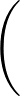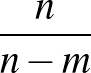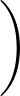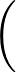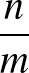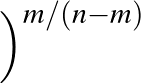ε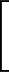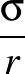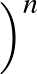-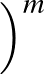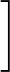.

The parameters n, m, ε, σ and coordinates of each Mie site must be provided in the file filename. The expected file format is:
Nsites    n    m    ε1    ...    εNspecies    σ1    ...    σNspecies
 x1 y1 z1xNsites yNsites zNsites

where ε and σ are given for each species (e.g. for nanoalloys). Mie field(s) can be smoothly truncated using the optional cut-off parameter Rc, which will trigger the usual Stoddard and Ford procedure:

φ(r)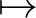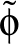(r) = φ(r) - φ(Rc) - (r - Rc)φ'(Rc).

Periodic boundary conditions with the nearest image convention can be imposed on the field sites using the optional parameters Bx, By and Bz, which specify the periodic box dimensions.

• MINBACKTCUT c: The internal coordinate back-transformation convergence cutoff used in all cases except for interpolations. Default value is 1.0×10-4

• MINMAX x: specifies the minimum value that the maximum step size is allowed to fall to. The default value is 0.01.

• MLMIN in start hidden out data λ min: calculates probability and classification properties for min neural network minima found already. Each neural network is an MLPVB3 network (see below), and the parameters have the same meaning. The weights of each neural network should be located in a file called MLMINweights, which has the format:  ... ...

• MLPNORM: Used in combination with any of the machine learning keywords. It specifies that the input features should each be rescaled by the mean of each feature. This process can help with convergence issues in machine learning. This keyword must be placed in the data file before the corresponding machine learning keyword, otherwise an error will be thrown.

• MLPVB3 in start hidden out data λ: specifies a perceptron neural network with one input layer of in input nodes, one hidden layer of hidden hidden nodes, and one output layer of out output nodes. data is the number of data points the model trains with, stored in a file called MLPData. This file should be formatted as follows:  y1 x11 x21 x31 ... y2 x12 x22 x32 ... y3 x13 x23 x33 ... ... yNdata x1Ndata x2Ndata x3Ndata ...
where yi is the outcome for data point i, and xji is the jth input feature for data point i. The features will be read starting from the feature in column start (ignoring the y column).

The hidden and output layers also have bias weights contributing to them. The activation function for the hidden layer is hyperbolic tan. The activation function for the output layer is just the sum. Overall, the raw contribution from data point d for output i is given by

 yid(;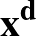) = wibo +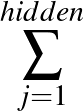wij(1)tanh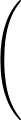wjbh +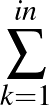wjk(2)xkd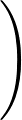(4)

These outputs become normalised softmax probabilities:

 pid(;) =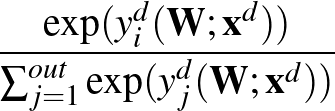(5)

The loss function to be minimised is:

 E(;) = -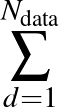ln pc(d)d(;) + λ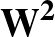, (6)

where c(d ) is the class label for data point d specified by the training set and λ is a constant coefficient of L2 regularisation, used to prevent overfitting. All weights are included in this L2 regularisation term, unless the keyword NOREGBIAS is present, in which case the bias weights are excluded from regularisation. The number of variables is hidden×(in+out+1)+out. The variables are ordered  wjk(2) input to hidden weights (iterating over inputs first), wij(1) hidden to output weights (iterating over hidden first), wjbh hidden bias weights, wibo output bias weights.

There are other, older keywords MLP3 and MLPB3, which were similar but had fewer weights. MLP3 had no bias weights. MLPB3 (now retired) was the same as MLP3 but with a single extra bias weight added before the tanh activation function in the hidden layer. Note that MLP3 and MLPB3 did not have the start parameter available to specify, and the MLPdata file had a slightly different format: features listed before outcomes.

• MLSUP in start hidden out data λ: specifies a perceptron neural network similar to the MLPVB3 keyword above. The parameters all have the same meaning as above. This keyword must come after the MLMIN keyword in the data file. The number of inputs, start position, and number of data points must be the same as those used for MLMIN. The number of hidden nodes and the value of λ may be different. The number of output nodes must be the same as the number of neural network minima given to MLMIN. Each output is the softmax probability that the neural network minimum corresponding to that output is the best one to use for a given data point. In this way, MLSUP creates a neural network of neural networks": overall there is a network of weights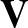used in a single-hidden-layered neural network, which are used to determine which neural network with weightsshould be used for a given data point.

• MODE n1 n2: specifies the eigenvector to be followed uphill in an eigenvector-following transition state search, where 1 means the softest mode, 2 means the next softest, and so on. Setting n1 to 0 means that the softest mode is followed uphill at every step, otherwise a maximum overlap criterion is used to determine the mode followed after the first step. The sign of n1 is used to determine the direction in which we step along the eigenvector if we are starting from a stationary point. This provides a way to start transition state searches from minima along any of the eigendirections in either sense. If a minimisation is started from a converged transition state then setting n1 to ±1 enables the program to walk down the two sides of the pathway. This is true for runs based on LBFGS techniques too if the keyword BFGSSTEP is used. MODE is now also used in EF/LBFGS searches so long as an analytical Hessian is calculated. n1 is used for the first step and n2 for subsequent steps. The default is 0 for both parameters. Note that n2 must be explicitly set equal to n1 to follow the same mode (as judged by overlap) in following steps.

• MODEDOWN n1 n2: specifies an eigenvector to be followed downhill in an eigenvector-following search, where 1 means the softest mode, 2 means the next softest, and so on. This keyword is intended for use with the INDEX keyword and SEARCH 2 setting. It enables us to step off a higher index saddle point and follow the corresponding mode downhill to search for a lower index saddle. If n2 is omitted it is set equal to n1 so that after the first step the downhill direction is judged by eigenvector overlap. The sign of n1 is used in the same way as for the MODE keyword to allow pushoffs in different directions along specifi modes.

• MORPH morphmxstp mnbfgsmax1 mnbfgsmax2 morphemax morpherise msteps: attempt to morph between endpoints by taking steps either towards or away from the endpoint specified in finish, and minimising in the tangent space. morphmxstp is the maximum step size. mnbfgsmax1 is the maximum number of minimisation steps taken in the tangent space when the distance from the finish endpoint is greater than the step size convergence criterion. mnbfgsmax2 is the maximum number of minimisation steps taken in the tangent space when the distance from the finish endpoint is less than the step size convergence criterion. morphemax is the maximum energy allowed during the interpolation; if this threshold is exceeded the algorithm toggles between stepping away/towards the finish endpoint. morpherise is the maximum energy rise permitted in a step. msteps is the maximum number of MORPH steps allowed. Used successfully with the general BLN model for protein L, where linear interpolation leads to strands crossing.

• MSEVBPARAMS shellsToCount maxHbondLength minHbondAngle OOclash_sq: specifies parameters for the MSEVB potential.

• MULTIJOB startfile finishfile: the same job will be rerun sequentially for input coordinates read from files startfile and finishfile. The usual coordinates are expected, for most systems after the POINTS keyword in odata, and the finish file, if applicable. After running this task, fresh coordinates are read from startfile and finishfile, if applicable to the run type. If the file names contain the string xyz' then the coordinates are assumed to be in xyz format. Otherwise they are read as an uninterrupted sequence of numbers in free format.

• MULTIJOB_MACHINE startfile ndof [finishfile] [i] [n] [step]: as multijob but the files containing restart coordinates are unformatted non-human-readable files. Each file contains one configuration per record. ndof is the number of degrees of freedom in the system, i.e. the number of entries in the record. i and n are the first and last records to read from the file, respectively. step is the interval between consecutive record numbers which will be read. So if i = 2, n = 10 and step = 3, the record numbers used to run OPTIM jobs will be 2,5,8. Default values of the optional parameters are NONE (i.e. no finish file required), 1, <eof>, 1. <eof> means the loop will run until the end of the input files. WARNING: fortran unformatted files may not be readable on a computer other than the one where they were produced, or if your OPTIM executable was compiled with a different compiler to the one which produced the unformatted file.

• MULTIPOT: Use the flexible multiple potential scheme to define your system. Different atoms can interact via different types of potential and a particular atom can be associated with more than one type of potential. Detailed instructions to use this scheme are given as comments in multipot.f90, pairwise_potentials.f90 and isotropic_potentials.f90.

In theory, any subroutine with the correct signature can be used as a potential function. Only the potentials in pairwise_potentials.f90 and isotropic_potentials.f90 have been tested. At the time of writing, these are the LJ, WCA and harmonic-spring potentials. pairwise_potentials.f90 contains functions which are called for a pair of atoms at a time (e.g. harmonic springs). isotropic_potentials.f90 contains functions which require coordinates of all the atoms involved in the interaction. Although LJ and WCA are pairwise potentials, it is more efficient to treat them as isotropic and loop over interacting pairs in the function call rather than to call pairwise_lj for every pair of atoms (N2 function calls give a large performance overhead).

pairwise_potentials.f90 and isotropic_potentials.f90 contain generic functions for calculating the first and second derivatives of isotropic pairwise potential functions. Detailed instructions are found in the source code.

An input file multipotconfig is used to specify which potential functions are to be used and which atoms use each potential. For each type of potential in the system, multipotconfig should contain the following:

• MULTIPULL npull: allow for multiple pulled pairs of atoms to be added through the PULL keyword. npull is the number of pairs added, where a separate entry with PULL is needed for everyone. This keyword has to be before the first entry for PULL, otherwise the arrays are not correctly used.

POT

POTTYPE n scale nparams

[params]

followed by a list of atom indices which will use this potential (the format of the list will depend on the particular potential).

POTTYPE is a string identifier for the type of potential being used, n is the number of atoms using this potential and scale is the energy unit for this potential (which should be set to 1.0 for at least one potential). nparams is the number of potential-specific parameters which are required, and [params] is a list of these parameters.

Be aware that using MULTIPOT will give a slight performance hit, because of the several function calls and bookkeeping that is associated with each call to POTENTIAL. This scheme is intended for playing around with composite potentials, for systems which are small enough that performance is not a big issue, or for systems involving many different potential functions which will be much too fiddly to hard-code a single subroutine.

• NAB inpcrd inpcrdformat: specifies a calculation with the interfaced version of the Nucleic Acid Builder program package. From this package the Amber force fields are being used only. The syntax is the same as for the AMBER9 keyword. For energy and gradient evaluations the standard Amber 9 interface is being called. The NAB routines are only called for analytical second derivative evaluations. The NAB interface does not have smooth cutoffs implemented at the moment, therefore this keyword should only be used for systems where the cutoff is large.

• NATB: specifies a tight-binding potential for sodium, silver and lithium.

• NATINT: use Pulay's natural internal coordinates[29,30] wherever internal coordinates are used. These coordinates can be generated automatically or with a parameter file (using the INTPARFILE keyword). If using the automatically generated coordinates, the user must specify separately any rings that are not part of PRO, PHE, TYR, TRP, or HIS residues (use the RING keyword).

• NCAP: calls the capsid model , consisting of pentagonal building blocks.

• NEB nsteps nimage rmsneb: specifies a nudged elastic band pathway calculation[31,32] using a maximum of nsteps steps with nimage images and RMS convergence criterion rmsneb. The default values are nsteps=100, nimage=10, rmsneb=0.1.

• NEBK nebk: specifies the value of the force constant for NEB and DNEB runs. The default is 100, which may be larger than the optimal value for some systems.

• NEBREADGUESS file : initial images for DNEB are read from file file in xyz format, as dumped by DUMPNEBXYZ, to allow for restart from the last step, if DUMPNEBXYZ 1 is set.

• NEBREDISTRIBUTE n : Redistribute NEB images evenly for current interpolation every n steps. A negative value for n does the initial interpolation as well, for use with READGUESS.

• NEWCONNECT NConMax NTriesMax ImageDensity IterDensity ImageMax ImageIncr RMStol: specifies the new connection algorithm described by Trygubenko and Wales. A finish file containing the coordinates of a second minimum is required in the current working directory, as for CONNECT. NConMax is the maximum number of cycles allowed, default=100. NTriesMax is the maximum number of connection attempts for any pair of local minima in the stationary point database, default=3. ImageDensity is a real variable specifying the number of DNEB images to be used for the first connection attempt for any pair of minima, default=10.0. An image density is specified, so that the actual number of images is ImageDensity×the separation between the two minima. If NTriesMax> 1 then the image density is increased by ImageIncr for each attempt, up to a maximum of ImageMax images in total. The default values for ImageIncr and ImageMax are 0.5 and 400, respectively. IterDensity specifies the maximum number of iterations used to optimise the DNEB in terms of a density. The actual value used is IterDensity×the number of images. The default value of IterDensity is 30.0. RMStol is the convergence condition for the RMS DNEB force on all the images. See also DNEBEFRAC. NEWCONNECT runs can be restarted and continued using the REDOPATHXYZ keyword. All path.n.xyz files numbered sequentially from 1 will be read, along with a pairs.newconnect file (optional) to provide the history of connection attempts. Make sure the number of NEWCONNECT cycles is large enough to read all the path.n.xyz files and continue as required.

• NEWNEB Nimage NIterMax RMSTol: specifies that the DNEB algorithm should be used for a double-ended transition state search. Nimage, NIterMax and RMSTol are the number of images, the maximum number of iterations and the RMS force convergence condition, respectively. The default value for Nimage is 2 and there are no defaults for the other parameters. If NEWCONNECT is also specified then these values are used for the first NEWCONNECT cycle only. However, if CONNECT is specified they are used for every cycle. See also DNEBEFRAC.

• NGUESS n: n is the number of transition state guesses to be tried in guessts for CHARMM or UNRES before switching back to NEB or NEWNEB.

• NOCHIRALCHECKS: disables checks for inversion of CA atoms and chiral side-chains for ILE and THR.

• NOCISTRANS minomega: used in CONNECT and NEWCONNECT runs to reject transition states that connect two minima with different omega angles, i.e. to prevent cis-trans peptide isomerisation. Checks that all residues besides proline are in the trans state and rejects transition states and minima that are not, i.e. with | ω| <minomega. minomega defaults to 150 degrees. For proline every ω is allowed. NOCISTRANS is the default for CHARMM, AMBER9 and NAB, i.e., no need to specify this line in the odata file unless you want to change minomega. If you want to allow for cis-trans isomerisation, you have to specify the CISTRANS keyword.

• NOCUDALBFGS: do not use the CUDA LBFGS routine. If CUDA A is present, the wrapper for AMBER CUDA energy and gradient will still be used, and the CPU version of LBFGS. This setup enables other terms to be added to the potential, e.g. using the PULL keyword.

• NOFRQS: specifies that frequencies are not present in path.info files. Used for very large systems where frequency calculations are not feasible in conjunction with the same PATHSAMPLE keyword.

• NOHESS: no Hessian should be calculated during geometry optimisation. Hybrid EF/LBFGS transition state searches are therefore conducted without a Hessian. The smallest eigenvalue and the corresponding eigenvector are found from a separate Rayleigh-Ritz minimisation problem for every combined EF/BFGS step.

• NOINTNEWT: when doing the back transformation from internal to Cartesian coordinates, solve the linear problem only. Do not do the Newton's-method iterations. This was the default in previous versions of OPTIM.

• NOINVERSION: turns off inversion of structures for distance metric in minpermdist.

• NOIT: for use with hybrid eigenvector-following runs when a Hessian is available. Specifies that the required lowest eigenvalue and eigenvector be found using LAPACK routine DSYEVR instead of by iteration.

• NOLBFGS: For the growing string or evolving string double-ended transition state search methods, instead of using L-BFGS optimisation to evolve the strings, simply take steps in the direction of the perpendicular force.

• NONEBMIND: do not put end point structures supplied to DNEB into closest coincidence.

• NONLOCAL x y z: factors for averaged Gaussian, Morse type 1 and Morse type 2 potentials.

• NOPOINTS: printing of coordinates to file points for intermediate steps is turned off. The default is true.

• NORANDOM randomcutoff: used in CHARMM transition state guessing procedure together with TWISTTYPE. Setting randomcutoff very large prevents random steps, and is recommended.

• NORESET: specifies that periodic images should not be returned to the primary supercell for calculations involving periodic boundary conditions. (May be useful for calculating transport properties with kinetic Monte Carlo).

• NOTE: has the same effect as COMMENT, i.e. the rest of the line is ignored.

• NOTRANSROT: forbid overall translation/rotation in alignment and distance calculations.

• NTIP 4: calls the TIP4P model of water molecules.

• ODIHE: for use with CHARMM. Specifies calculation of a dihedral-angle order parameter using the reference structure supplied in ref.crd.

• OH: add an octahedral field of strength x to the potential.

• OHCELL : allow point group operations for a cubic supercell in subroutine minpermdist.f90 permutational distance minimisation.

• ONETEP job system: tells the program to use a ONETEP executable to calculate energies and forces with input files based on the system name system. Periodic boundary conditions are not assumed, so projection or shifting is performed to remove overall translation and rotation. job is a string in quotes that specifies the system call required to run the ONETEP executable. The line print_qc : TRUE' is needed in the system.dat file so that the total energy is printed in a form that OPTIM can grep for. OPTIM will change the line with read_denskern' in the system.dat file to TRUE after the first call to obtain the ONETEP energy and gradient. This saves a great deal of cpu time in subsequent CASTEP steps. However, it means that the final dat file is altered, and you will need to change read_denskern to FALSE to restart the job if a valid system.dkn file is not present in the working directory. ONETEP will fail and stop if the wavefunction read fails. Note that you may not be able to restart a job with a different number of nodes using a saved density (not tested). The PARALLEL keyword is not needed, since the number of processors can be specified in job if necessary. Example for volkhan:

ONETEP 'mpirun /home/wales/bin/onetep' 'ethene'

For a CASTEP or ONETEP normal mode analysis set STEPS 0, MASS, ENDHESS, and ENDNUMHESS in odata. The Hessian eigenvalues and normal mode frequencies are printed to standard output automatically. Check pertable.f first to make sure that the atomic mass is known for all the elements in your system. The frequency conversion assumes that the units of energy and distance are electron volts and Å. If DUMPVECTOR ALLVECTORS is set in odata then all the normal mode eigenvector components will be transformed to the Cartesian basis from the mass-weighted Cartesian basis.

• OPEPHIRE option start: alternative use OPEP or HIRE, specifies the use of the HiRE and OPEP potentials, option is Protein, RNA, DNA, MixedRNA or MixedDNA. The following additional files are needed for OPEP protein simulations: conf_initiale.pdb, ichain.dat, scale.dat, cutoffs.dat, parametres.top and parametres.list. For RNA and DNA simulations the following files are required: conf_initiale.pdb, conf_initiale_RNA.pdb, ichain.dat, scale_RNA.dat, cutoffs_RNA.dat, parametres_RNA.top, baselist.dat, bblist.dat, chargeatm_RNA.dat and protonated.dat. Coordinates for the potential are provided by start and finish.

• OPTIMIZETS: optimise transition states at the end of each DNEB run. Default false. Set to true by NEWCONNECT.

• ORBITGEOMTOL x: sets the cutoff value for assigning atoms to the same orbit when analysing the standard orientation in routine myorient. The default value for x is 0.3. If x is too small it is possible for permutational isomers to be missed.

• ORT: remove overall rotation and translation for DNEB runs. Default false.

• OSASA rpro: for use with CHARMM. Specifies calculation of a solvent accessible surface area order parameter using probe radius rpro.

• PAHA n: calls a finite system of polycyclic aromatic hydrocarbons (PAH) interacting via a general anisotropic potential developed from first principles . The PAH ID n defines the PAH molecule: 1 for benzene, 2 for naphthalene, 3 for anthracene, and 4 for pyrene.

• PAP npatch alpha s cosdel epsilon: specifies a patch anti-patch potential. Each body consists of a Lennard-Jones core, range parameter alpha, with npatch patches and npatch anti-patches. The patch anti-patch attraction has a range specified by s, a width specified by cosdel and a strength specified by epsilon. If npatch is set to zero, site information will be read in from the file papsites.xyz.

• PARALLEL nproc: specifies that auxiliary programs such as GAMESS and CPMD use nproc processors. Not required for CASTEP.

• PARAMS: enables additional parameters to be set for specific potentials, e.g. Z* for LJAT, ρ0 for Morse, n, m, the box lengths Lx, Ly and Lz and the cutoff for ME, the box lengths Lx, Ly and Lz and the cutoff for JM, SC and P6, and ε, c and σ for Au, Ag and Ni. Note that all the numbers are read as real.

• PATH n de ds: requests calculation of the pathway connecting two minima from the transition state specified in odata. n is the number of intermediate points files to save on either side, default n=3. To choose which frames are dumped in the path.xyz file a stochastic method is employed. All three stationary points are always included. Other frames are dumped with a probability proportional to n. If n exceeds the number of frames in the path then they will all be dumped, up to a maximum of 100. This behaviour can be modified by specifying the optional parameters de and ds. These are thresholds for the energy change and path length change required for the next frame to be included. The defaults are both zero. The complete xyz file is printed to path.xyz and the energy as a function of path length is printed to file EofS. A summary of the path characteristics such as barrier heights, path lengths and cooperativity indices is printed at the end. If READVEC is present in odata then the required eigenvector corresponding to the displacement direction will be read from vector.dump, otherwise it will be calculated by diagonalizing a Hessian (for SEARCH 2) or using an iterative approach (for BFGSTS with or without NOHESS; NOIT can also be used with BFGSTS.) After the initial step the energy will be minimised by whichever first or second order method is specified in odata, i.e. eigenvector-following or second-order Page-McIver for SEARCH 0 and 6 and 7, LBFGS for BFGSMIN, and first order steepest-descent for BSMIN and RKMIN. A hybrid method can be specified using HYBRIDMIN. See also DUMPPATH and PATHSDSTEPS..

• PATHSDSTEPS n: specifies the maximum number of minimisation steps in a pathway calculation before switching to LBFGS. Can be used with search types

• PBS : for use with MCPATH2; distribute sampling for independent blocks over as many cores as specfied by the qsub script for PBS.

• PERMDIST x nsec: minimise distances between stationary points with respect to permutational isomerisation. Requires the auxiliary file perm.allow to specify permutable atoms, otherwise all atoms are assumed to be permutable. The absence of a perm.allow file is considered a mistake for CHARMM runs.

The first line of the perm.allow file must contain an integer that specifies the number of primary groups of interchangeable atoms. The groups then follow, each one introduced by a line with two integers p and s that specify the number of permutable atoms in the primary group and the number of other sets of permutable atoms associated with the primary set. When a permutation is applied to the primary set, the same permutation is applied to the atoms in the secondary set. The secondary sets must all be the same size as the primary set, i.e. p members. s may be zero; this is the usual case. The following line contains the indices of the p permutable atoms in the primary set and then the indices of the atoms in each of the s secondary sets, one set at a time.

The parameter x is the distance tolerance for assigning atoms to orbits in the myorient standard orientation routine, default value 0.3. If x is too small it is possible for permutational isomers to be missed. The parameter nsec specifies the maximum number of atoms permitted in secondary sets. The default is 3, which is sufficient for methyl groups in bio-molecules. Rigid bodies may require a higher number.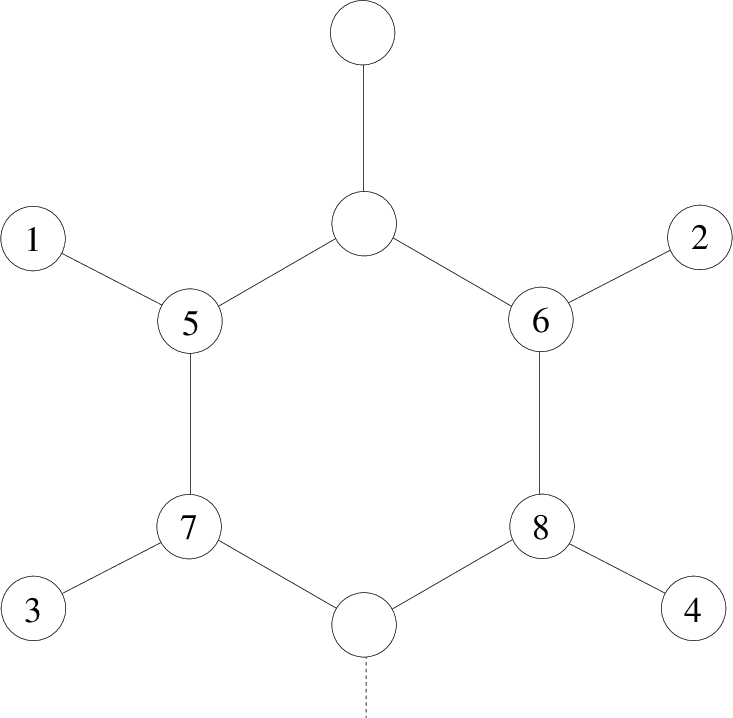For the phenylalanine example illustrated above we must allow three other pairs of atoms to exchange if we swap 7 and 8. Hence a suitable perm.allow entry is

1

2 3

7 8 5 6 1 2 3 4

This should be on three consecutive lines. Here n = 2 and s = 3: if we exchange 7 and 8 then we must also exchange 5 and 6, 1 and 2, and 3 and 4. There are two atoms in each of the three secondary sets, since we have specified 7 and 8 as the two primary atoms.

Here is an example perm.allow file for a water trimer using the flexible QTIP4PF potential, where the energy is invariant to permutations of water molecules and to exchanges of hydrogens in the same molecule. However, hydrogens cannot exchange between different oxygens:

4

3 2

1 4 7 2 5 8 3 6 9

2 0

2 3

2 0

5 6

2 0

8 9

The first group of three oxygens has two atoms that must move with each oxygen, i.e. atoms 2 and 3 for oxygen 1, etc. Hydrogen permutations for each oxygen are allowed by the three following groups. This scheme allows atoms to appear in more than one group. There must be a group containing each complete set of permutations in order for permutation-inversion isomers to be recognised. The format is compatible with an older scheme, where only pair swaps were allowed for associated atoms, but now allows for more general permutations.

Scripts to generate allowed permutations automatically for CHARMM and AMBER are available from the group web site. It is essential to use symmetrised versions of the corresponding force fields!

• PERMDISTINIT x: performs the permutational analysis described above for PERMDIST, but only for the initial alignment of two end points. After this is done, the permutational optimisation is turned off, which can save time.

• PERTDIHE chpmax chnmin chnmax iseed: CHARMM and UNRES dihedral angle perturbation specification. Performs random, GMIN-style twists before starting optimisation.

• POINTS: this is usually the last keyword and introduces the coordinate information, which is read one atom per line. Each line must consist of the atomic symbol of the atom followed by its three Cartesian coordinates. Leading spaces before the atomic symbol should be avoided. For calculations involving CHARMM, UNRES, AMBER and CPMD the coordinates are obtained from auxiliary files, and POINTS will be the last OPTIM directive in the odata file, or is not used (CHARMM and UNRES).

• PMPATH inr: calculates the approximate steepest-descent pathways leading from a transition state with the Page-McIver second order formulation. inr can be 6 or 7, and has the same effect as the corresponding settings for the SEARCH keyword. inr=6 should only converge to minima (default), while inr=7 produces paths that can terminate at saddle points.

• PREROTATE: For the GS and ES double-ended transition state search methods, if using FIXATMS to zero some coordinates of the forces to avoid overall translation and rotation, this keyword will rotate the start and end points so that those coordinates are zero in both. The default is true.

• PRESSURE: if present tells the program to perform a constant zero pressure optimisation for bulk SC, ME, MP, MS and P6. Normally a constant volume optimisation takes place.

• PRINT n: sets the print level. The default value is zero. Use of this option is not recommended, since more specific print control is possible.

• PRINTCOEFFICIENTS: prints ground state coefficients for the MSEVB potential.

• PTSTST npatch eps cosdel kappa Specifies a patchy site-to-site potential, similar to PAP but with Gaussian patches on the surface of the particle. The number of patches is determined by npatch. eps is the strength of the Gaussian patch attraction and cosdel is the effective range of the Gaussian.

• PULL a1 a2 f: apply a static force to the potential, equivalent to adding the term Vpull = - f (za1 - za2). Here za1 and za2 are the z coordinates for atoms a1 and a2, and f specifies the force. This potential is designed to simulate a pulling experiment with static force where a molecule is pulled along the z axis from atoms a1 and a2.

• PUSHCUT x: sets the threshold for the RMS force below which the system may apply a PUSHOFF to escape from a stationary point of the wrong Hessian index. Default value is 0.00001. Using a negative value prevents pushoffs from occurring.

• PUSHOFF x: sets the magnitude of a step away from a stationary point of the wrong Hessian index (see §8 for default action). Default value is 0.01. Formally used as the step size in hybrid eigenvector-following transition state searches if the eigenvalue of the direction being followed is positive. MAXMAX has now replaced PUSHOFF in this role.

• PUSHOPT x conv max: specifies that the magnitude of steps away from a transition state in path calculations should be calculated using a golden section search in the interval [0 : x] and [0 : - x] for the tw directions. The search terminates when the pushoff magnitude for the lowest energy in the line search is identified to an accuracy of conv, or when max iterations have been performed.

• PV press gtol grad steps: specifies an approximate constant pressure optimisation with pressure press and convergence criterion gtol for the gradients with respect to the box lengths. If any box length gradient is larger in magnitude than grad then up to a maximum of steps optimisation steps for the box lengths are performed. If the three box lengths are equal to start with and the keyword CUBIC is included, then a cubic box is maintained. Will not work with atom types MP, ME and MS. Default values, press=0, gtol=0.001, grad= 1060, steps=100.

• PVTS press gtol grad nboxts: as for PV but searches for a transition state with respect to box length nboxts, where nboxts=1, 2 and 3 specify the x, y and z box lengths, respectively. nboxts defaults to 1.

• PYG a1 a2 a3 b1 b2 b3 sigma0 eps0: calls a finite system of Paramonov-Yaliraki ellipsoids , where the potential involves a set of eight parameters, six of them, {ak} and {bk} (k = 1, 2, 3), defining two different shape matrices for the repulsive and attractive parts of the interaction in terms of the lengths of the semi-axes, and the other two, σ0 and ε0, defining the length and energy scales, respectively.

• QCHEM qchemjob sys: calculate energy and gradient using the QCHEM program. qchemjob is a string specifying how to run the QCHEM executable, and sys is a string specifying the QCHEM input file.

• QCHEMSCF qchemjob sys nao nmo nalpha nbeta: specifies a QCHEM job to search the SCF solution landscape. qchemjob is a string specifying how to run the QCHEM executable, as for conventional geometry optimisation, and sys is a string specifying the QCHEM input file. nao nmo nalpha nbeta are the number of atomic orbitals, molecular orbitals, alpha spin, and beta spin electrons, respectively. The initial molecular orbital coefficients are read from the file start. The output file qcoverlap is needed in the current working directory for PATHSAMPLE to calculate distance metrics between solutions. An example job for the H4 molecule with a 3-21G basis could have
QCHEMSCF 'qchem -save -nt 1' 'h4' 8 8 2 2
and a QCHEM input file h4 containing $comment H4 HF 3-21G Single point energy$end

$molecule 0 1 H 1.00000 1.000000 0.00000 H 1.00000 -1.000000 0.00000 H -1.00000 -1.000000 0.00000 H -1.00000 1.000000 0.00000$end

$rem method hf Exact exchange BASIS 3-21G Basis Set SCF_CONVERGENCE 8 Tight convergence sym_ignore true unrestricted true SYMMETRY FALSE SCF_MAX_CYCLES 0 GEN_SCFMAN TRUE SCF_GUESS READ$end This system has 128 molecular orbital coefficients but only 24 variables. Note that QCHEM performs zero SCF cycles because we seek the energy for a given set of molecular orbital coefficients, and derivatives with respect to the degrees of freedom specified by a constraint surface (Thouless transformation). With BASIS aug-cc-pVDZ the odata line is
QCHEMSCF 'qchem -save -nt 1' 'h4' 36 36 2 2
and there are 2592 molecular orbital coefficients and 68 variables.

• QCHEMGHF qchemjob sys nao nmo nelec: specifies a QCHEM job to search the SCF solution landscape for the GHF approach. qchemjob is a string specifying how to run the QCHEM executable, as for conventional geometry optimisation, and sys is a string specifying the QCHEM input file. nao nmo nelec are the number of atomic orbitals, molecular orbitals, and electrons, respectively. The initial molecular orbital coefficients are read from the file start. The output file qcoverlap is needed in the current working directory for PATHSAMPLE to calculate distance metrics between solutions. An example job for the H4 molecule with a 3-21G basis could have
QCHEMSCF 'qchem -save -nt 1' 'h4' 8 8 4
and a QCHEM input file h4 containing $comment H4 HF 3-21G Single point energy$end

$molecule 0 1 H 1.00000 1.000000 0.00000 H 1.00000 -1.000000 0.00000 H -1.00000 -1.000000 0.00000 H -1.00000 1.000000 0.00000$end

$rem method hf Exact exchange BASIS 3-21G Basis Set SCF_CONVERGENCE 8 Tight convergence sym_ignore true unrestricted true SYMMETRY FALSE SCF_MAX_CYCLES 0 SCF_GUESS READ GEN_SCFMAN TRUE SCF_SAVE_HESSIAN TRUE$end Note that, as for QCHEMSCF, QCHEM performs zero SCF cycles because we seek the energy for a given set of molecular orbital coefficients, and derivatives with respect to the degrees of freedom specified by a constraint surface (Thouless transformation). This system has 256 molecular orbital coefficients but only 48 variables.

• QCI or INTCONSTRAINT intconstrainttol intconstraintdel intconstraintrep intconstrainrepcut intconfrac intconsep intrepsep intsteps1 intconsteps intrelsteps maxcone intgradtol specifies quasi-continuous interpolation via an auxiliary constraint potential. intconstrainttol is used to determine constrained distances. The deviation of the distance between a given pair of atoms in all reference structures from the average must be less than intconstrainttol, default 0.1, for this separation to constitute a constraint. If a percolating network of constraints does not result then intconstrainttol is increased by 10% and the analysis repeated. This parameter is not used if QCIAMBER is set, since the bond length and angle constraints are set automatically, and additional planarity constraints can be read from the file constraintfile. This file can be generated using the program aaextraconstraint.f90 located in the svnSCRIPTSOPTIM directory, which requires a pdb file called coords.inpcrd.pdb in the current working directory. The list of backbone atoms for keyword DOBACKALL is also generated in file aabk by the aaextraconstraint.f90 program. intconstraintdel multiplies the constraint penalty term in the auxiliary potential, default 1.0. intconstraintrep multiplies the repulsive penalty term in the auxiliary potential, default 1.0. A dimensionless form of the penalty function is now used, so these factors only have an effect if they are set to zero to ignore one set of terms. intconstrainrepcut is the cutoff for the repulsive penalty term in the auxiliary potential, default 1.7. intconfrac is the fraction of the true potential used for the second optimisation phase after the interpolation with the constraint potential has been achieved. The default value is 0.9. intconsep is the maximum difference between the order number of atoms for which constraints are allowed. The default is 15, which seems appropriate for biomolecules. However, if a congeom file of reference minima is specified, or a corresponding congeom.dat file is present, or if QCIAMBER is set, then intconsep can be set greater than the total number of atoms. intrepsep is the minimum difference in order number of atoms for which repulsive terms are added to the potential. The default is zero; there are no repulsive terms between constrained atoms. intsteps1 is the maximum number of minimisation steps allowed for optimisation of the interpolation potential, default 300000. intconsteps is the number of additional minimisation steps after interpolation with the auxiliary potential has been achieved, where the potential is changed to include a fraction intconfrac of the true potential, default 100. intrelsteps is the number of minimisation steps allowed after adding an atom in the interpolation phase before a new atom is added, even if the convergence conditions on the interpolation metric and root mean gradient have not been achieved, default 200. In the current setup if QCIRESET is used then a much larger value may be better so that atoms are not added until a convergence condition is hit. maxcone is the convergence threshold for the highest value of the auxiliary constraint or repulsive potential for a single atom, default 0.01. intgradtol is the convergence threshold for the highest absolute value of the auxiliary constraint or repulsive potential for a single atom, default 0.01.

Any number of reference minima can be specified in the additional file congeom in the current working directory. If this file is found then the constraints and repulsive terms are fixed using these minima, and a summary of all the terms required for the interpolation potential is written to file congeom.dat. If neither file is found then the interpolation potential is based on the two end points only, unless QCIAMBER is employed with a separate constraintfile, which is strongly recommended. and it may be necessary to set intconsep. All geometries are permutationally aligned in defining the interpolation potential, using e.g. PERMDIST or LOPERMDIST. When congeom or congeom.dat is used all minima are permutationally aligned to the first minimum in the reference set. See also CONINT, CONCUTABS, CHECKREP, INTFREEZE, INTIMAGE, INTSPRINGACTIVE, KINT, MAXCON, QCIADDACID, QCIADJUSTK, QCICONCUT, QCIIMAGE, QCIIMAGEDENS, QCICYCLES, QCIDOBACKALL, QCILPDCON, QCILPERMDIST, QCIMAXACTIVE, QCIRESET, QCISTOP, QCITRILAT.

• QCIADDACID: specifies that the QCI routine should add atoms for each amino acid residue in turn, until it hits one with fewer than three active constraints. Atoms are added individually as normal once a pass through all residues has been completed. This option is not recommended.

• QCIALIGN: species a list of atoms for the initial end point alignment of end point minima. The list is read from file QCIalign, where the first line must contain the number of entries to be read.

• QCILINEAR: species a list of atoms that should have linear interpolation applied from the start of the QCI procedure. The list is read from file QCIlinear, where the first line must contain the number of entries to be read.

• QCIDOBACKALL: specifies that the QCI routine should add all backbone atoms for each amino acid in turn, including the atoms connected to the Cα carbon. Atoms are added individually as normal once a pass through all residues has been completed. The backbone atom file aabk is required in the current working directory, generated by aaextraconstraint.f90 (located in the svnSCRIPTSOPTIM directory).

• QCIFREEZE or INTFREEZE x specifies the root mean square force on a QCI interpolation image below which it is frozen when INTCONSTRAINT is used. The default value for x is 0.001.

• QCIRESET qciresetint: if the number of QCI steps exceeds qciresetint since the last atom was added, or since the last spring constant reset, or the last change in a permutational group or chiral inversion, then slacken the convergence criteria. Either or both of the QCI convergence parameters is increased by 5%, depending on which appear to be too tight.

• QCIADJUSTK qciadjustjfrq qciadjustktol qciadjustkfrac qcikintmin qcikintmax: increase or decrease the QCI spring constant KINT every qciadjustjfrq (default 0, no adjustment) steps by a factor of qciadjustkfrac (default 1.05) according to whether the percentage distance deviation of the images from the mean spacing is greater or less than qciadjustktol (default 10.0), respectively. The last two parameters specify lower and uppoer bounds on the spring constant, default 0.01 and 100. This keyword is analogous to ADJUSTK for the DNEB procedure.

• QCILPERMDIST qcipdint qcipermcut: for QCI interpolations, check the permutational alignment using the local permdist routine at intervals of qcipdint with alignment tolerance of qcipermcut. Atoms are permuted if the alignment agrees for the two fixed end points. This approach is the same as the normal local permutational alignment scheme, but applied to one permutational group at a time. The cutoff for accepting new atoms into the environment may need to be larger than usual, because QCI images may have more distorted geometries.

• QCILPDCON qcipdint: for QCI interpolations, check the permutational alignment using the local permdist routine based on all active atoms constrained to all the atoms of the permutational group at intervals of qcipdint. Atoms are permuted if the alignment agrees for the two fixed end points. For some groups, such as NH3+ in LYS and NH2 is ASP, there is axial symmetry, and alignment may be ambiguous. In this case we take the permutation with the fewest atom moves for alignments within GEOMDIFFTOL.
• INTSPRINGACTIVE: for QCI procedure, only apply spring terms for active atoms. Default

• QCIMAXACTIVE imaxnactive: for QCI interpolations, only allow imaxnactive active atoms. The active atoms drop off the list according to when they were first added. Probably best not to use this feature, so set imaxnactive greater than the number of atoms.

• QCICYCLES qcicycdist qcicycn: for QCI interpolations, only use QCI for gaps larger than qcicycdist and for cycles up to qcicycn

• QCICONCUT intconcut : for QCI interpolations, don't allow constraints between atoms separated by more than intconcut in either endpoint, default 100.0. INTCONCUT is the same.

• QCIIMAGE or INTIMAGE imsepmin imsepmax intimage maxintimage intntriesmax intimageincr intimagecheck specifies the behaviour of interpolation images for the INTCONSTRAINT quasi-continuous interpolation procedure. intimagecheck (default 25) is the interval for checking the spacing of the interpolation images. Images separated by less than imsepmin (default 0.2) can be combined, while an additional image can be added between images further than imsepmax (default 10.0) apart. intimage is the initial number of images (default 3), maxintimage is the maximum number of images (default 75), intntriesmax is the maximum number of quasi-continuous interpolation attempts for a given pair of minima (default 2), and intimageincr (default 6) is the increment in the number of initial images for further connection attempts between minima that have already been tried. It may be better to use QCIIMAGEDENS, documented below.

• QCIIMAGEDENS density max: use a fixed number of QCI images determined as the end point distance times the density parameter, with a maximum number of images max.

• QCMODE n: specifies how the user wants to distinguish between various solutions obtained in the hybrid QChem and OPTIM version. The four modes available are mode 0 , 1 , 2 , 3 and 4. Mode 3 is preferred over other modes because mode 0 and 4 undersample the space mode2 might mix in solutions that aren't relevant. The solutions obtained are differentiated on the basis of their orbital coefficients.

• QTIP4PF: flexible water potential from Habershon et al. [J. Chem. Phys., 131, 024501 (2009)] coded by Dr Javier Hernández-Rojas. The units of length and energy are Å and kcal/mol. The atoms must be entered in the order O, H, H, O, H, H, etc.

• QSPCFW: flexible water potential from Paesani et al. [J. Chem. Phys., 125, 184507 (2006)] coded by Dr Javier Hernández-Rojas. The units of length and energy are Å and kcal/mol. The atoms must be entered in the order O, H, H, O, H, H, etc.

• QUIPZ z: define the atomic number z for the quip interface.

• QUIPLATT matrix: defines the components of a 3×3 lattice for the quip interface.

• QUIP_PARAM_FILE qpf: sets the quip parameters file. qpf is a string and the default is quip_params.xml.

• QUIP_INIT_ARGS_STR ias: sets the quip parameter init_args_str'. ias is a string with default value xml_label=default'.

• RADIUS x: specifies that atoms should not be allowed to move beyond a distance x from the origin.

• RANROT n: for use with PERMDIST. Specifies that n random orientations will be tried as well as the initial standard alignment in minpermdist.f90. Several hundred random orientations may be needed to find the best translational/rotational/permutational alignment of end points.

• RANSEED n: specifies the initial seed for random number generation, as used in hard sphere moves with FIXD.

• RATIOS: specifies that the pathway parameters needed to calculate catastrophe ratios should be printed in a PATH run.

• RBSYM: specifies that internal symmetry operations permuting equivalent sites for rigid-body building blocks exist. The operations are read in from a file rbsymops, which must exist in the working directory. The first line of the file is an integer that specifies the number of operations generated by proper axes of rotation including the identity operation; the operations are then read in one at a time in a representation consisting of a unit vector, defining the axis in the reference frame, and an angle in degrees, describing the magnitude of rotation about that axis. The first operation has to correspond to the identity operation.

This keyword has to be combined with PERMDIST so that structures are aligned and a distance metric considered based on permutations of identical rigid bodies and any internal symmetry operation of each rigid body that is a symmetry of the potential energy function.

• READMASS: atomic masses will be read from file masses in the current working directory. This option may be useful for normal mode analysis with potentials such as binary Gupta.

• READPATH: if specified with CALCRATES then rate constants will be calculated for the transition states found in path.info and no stationary point searches will be performed.

• READSP: instructs OPTIM to read minima and transition state data in the PATHSAMPLE format. This has not been implemented yet!

• READHESS: Hessian will be read from file derivs at first step.

• READVEC: if present the Hessian eigenvector corresponding to the smallest eigenvalue will be read from file vector.dump. The latter file can be generated using the DUMPVECTOR keyword in a previous run.

• NEWREAXFF: specifies the use of the REAXFF force field. The following additional files are needed: fort.3 or start, which contain the starting geometry, fort.4, which contains a specific force field parametrization, control, which contains additional force field settings. We recommend using ReaxFF with a start file for the coordinates, in which case fort.3 will only be used to read atomic symbols and number of atoms in the system. The format of fort.3 is BGF and is format-sensitive.

• REAXFFDECOMP: print energy decomposition file (fort.200) for REAXFF.

• REDOPATH: instructs OPTIM to read transition state coordinates successively from the file redopoints during a NEWCONNECT run. Can be used to generate movie files from stationary point sequences calculated by PATHSAMPLE. The correct start and finish coordinates for the minima must be present in the odata and finish files. The odata file should contain a PATH keyword with sufficeint frames to make a movie. Just using PATH with no arguments will just dump the three stationary points in each case, which were already known from the input. The number of NEWCONNECT cycles must be greater than the number of transition states in the path.

There can often be problems for long paths if a transition state does not manage to match the two minima in the dicrete path that has been read in. OPTIM will try different parameters for the path run in this case, and will continue to try and bridge any gaps that remain when the pathdata file is exhausted, if there are additional NEWCONNECT cycles available.

Each transition state should already be converged, and the transition state searches should converge immediately. However, in a gradient-only BFGSTS run, the initial eigenvector guess is random to begin with, so more Rayleigh-Ritz steps may be needed. It is a good idea to increase this maximum value on the BFGSTS line.

• REDOPATHXYZ: as for REDOPATH above, except that existing path.n.xyz files are read instead of actually calculating pathways for the transition states. NEWCONNECT runs can be restarted and continued using the REDOPATHXYZ keyword. All path.n.xyz files numbered sequentially from 1 will be read, along with a pairs.newconnect file (optional) to provide the history of connection attempts. Make sure the number of NEWCONNECT cycles is large enough to read all the path.n.xyz files and continue as required.

• REDOTS n: The CONNECT algorithms often find the same refined TS multiple times. This keyword specifies that the first n times a TS is found, the pushoff path is calculated as normal. Subsequent times that the TS is found, the path is not calculated and the TS simply ignored. n has default value 2 (i.e. one re-try of the path run).

• REDUCEDBONDLENGTH blfactor CB: rescales the bond lengths by a factor of blfactor in the side chains of proteins, if modelled by one of the CHARMM potentials. blfactor has to be specified, and its default value is one. CB is an optional argument. When set, the bond length between the Cα and Cβ atoms is rescaled by blfactor as well, otherwise not.

• REOPT: specifies that the eigenvector to be followed should be reoptimised in a BFGSTS search after the EF step and before the tangent space minimisation. This is probably not a good idea. Default is false.

• REOPTIMISEENDPOINTS: specifies that endpoint minima should be reoptimised if their RMS force lies above the convergence threshold. Useful in connection runs where failure is guaranteed if one or more of the endpoints is not identified as a minimum. A large RMS force at the beginning of a connection run driven by PATHSAMPLE should be investigated because it suggests a problem, e.g. with CHARMM permutational isomerisations. Default is false.

• REPELTS: specifies that the transition state search direction should be orthogonalised to the coordinates in file points.repel.

• RESIZE x: scales all the coordinates by x before the first step is taken.

• REVERSEGEDIFFTOL: Reverses the order of comparison between two structures (minima and transition states). First, their cartesian distance will be calculated and compared. If the distance is larger than GEOMDIFFTOL, the structures are different. If the distance is less than GEOMDIFFTOL, the energies of the two structures will be compared. If their energies differ by more than EDIFFTOL, the structures are considered different, or else identical. Typically, this leads to a slower calculation since the energies are already present, while the distance comparison requires additional calculation. Nevertheless, this is useful in cases where the energy landscape is noisy and has a very rough topography (i.e. structuraly very similar structures), which is sometimes the case for ReaxFF force fields. When using this keyword, it is also important to use MAXIMFACTOR in order to properly scale the factor used to identify local maxima for hybrid EF TS searches (It multiplies EDIFFTOL).

• RIGIDINIT AACONVERGENCE : This will turn on the local rigid body framework. This keyword needs two additional input files: coordsinirigid and rbodyconfig. coordsinirigid should contain the coordinates of all the atoms whether they are part of local rigid bodies or not. During initialization, OPTIM will select appropriate coordinates and throw away irrelevant ones. The format is:
x1 y1 z1
x2 y2 z2
ldots
ldots
xn yn zn

The file rbodyconfig defines the rigid bodies, with the following format:
GROUP NoAtomsInGroup
Atom 1
Atom 2
ldots
ldots
Atom N

This keyword takes an optional argument AACONVERGENCE . If present, this switches on the use of the more accurate distance measure and method of calculating the RMS force for angle-axis coordinates described in this paper: V. Ruhle, H. Kusumaatmaja, D. Chakrabarti and D. J. Wales, J. Chem. Theory Comput., 2013, 9, 4026-4034.

• RIGIDMOLECULES: For use only in conjunction with RIGIDINIT. Indicates a system composed of multiple rigid bodies and having no free atoms. This is a temporary keyword, used to isolate some parts of the rigid body code from the biomolecule potentials and similar. Most users probably don't need to use it, but talk to sn402 if you're unsure. Hopefully this keyword will soon be removed completely and its functionality absorbed into other keywords as appropriate.

• RING a1 a2...: When using natural internal coordinates, specifies a ring that's not part of a PRO, PHE, TYR, TRP, or HIS residue. a1, a2, etc. are the atom numbers for the ring in consecutive order. Only rings of size 5 or 6 are permitted.

• RINGPOLYMER rpsyst nrp rpbeta rptype: Specifies a ring polymer system with harmonic springs between nrp images of the same system that generally have different geometries. rpsyst is a string specifying the system: AECK (asymmetric Eckart barrier), SD (Stillinger-David flexible water potential), TT (Xantheas' TTM3-F water), MCY (VRT(MCY-5f) water), JB (James Bowman's water), MB (MBPol water).
nrp is the number of RP images. rpbeta is 1/kT in reduced units. rptype takes keywords of RPCYC (for ring polymer), RPLIN (for linear polymer) and RPFIX (for a linear polymer with fixed ends, provided in files xminA and xminB). RINGPOLYMER keyword takes the place of POINTS and must be the last keyword in the odata file before the points.

• RKMIN gmax eps: calculates a steepest-descent path using gradient only information with convergence criterion gmax for the RMS force and initial precision eps. A fifth order Runga-Kutta algorithm is used.

• ROT: if present a rotational kinetic energy term is added to the Hamiltonian. Either constant angular momentum (ROT JZ x) or constant angular velocity (ROT OMEGA x) may be used to specify Jz or ωz.

• RPPROJEXTRA: for RINGPOLYMER project out the coordinate corresponding to cyclic permutation of beads in the BFGSTS Rayleigh-Ritz procedure. This mode can produce an additional zero eigenvalue if the number of beads is large enough.

• SCALE n: sets the scaling algorithm for eigenvector-following steps, as described in §8. The default is n=10.

• SCORE_QUEUE: specifies an Score parallel environment. Currently not used on group clusters.

• SEARCH n: specifies the search type for eigenvector-following and steepest-descent calculations based on second derivatives and Hessian diagonalisation, default is type 0. The most common options are 0, a minimisation, and 2, a transition state search. See §5 for full details.

• SHIFT x: specifies the shift applied to eigenvectors corresponding to normal modes that conserve the energy. The default is 106. The shift must be large enough to move the eigenvalues in question to the top of the spectrum.

• SLERP: activates the iSLERP algorithm for rigid bodies when interpolating an initial band for the NEB. This algorithm (due to Li, X., J. Graphics Tools 12 (3), 1 (2011)) uses quaternions to provide an efficient route to smooth interpolation of a rigid body between two endpoints. Without this keyword, default behaviour is to interpolate rigid body coordinates as though they were cartesian. This is often not a problem, but can lead to uneven interpolation and occasionally very bad interpolated bands. The iSLERP algorithm seems to give reliably good interpolations.

• SPRING type limit factor: specifies the use of a surrogate spring potential. For ReaxFF (or other potentials) we may need to restrain some bonds to a specified value to avoid reactions. In such cases, the surrogate spring potential should be added between one or more pairs of atoms in the system. type specifies the type of spring: either harmonic or reaxff. limit (neb or none) specifies whether we should limit its operation. Currently, the surrogate potential can be limited to operate only during a neb stage or without any limitation whatsoever, i.e. none. factor (default: 0.95) specifies a value that multiplies the spring constant(s) in each call to potential. The lowering of spring constant(s) can be used to recover the original potential at the end of the NEB stage. A file called springfile is required to specify the pairs of atoms to be restrained together with the associated spring constants and bond length restraints. The syntax of springfile is the following:
N
Ati Atj Kij Rij

Where N is the total number of listed bonds, Ati and Atj are atom ID's, Kij is a spring constant and Rij is a bond restraint length. In case of a reaxff type spring, an additional spring constant should be listed immediately after the 1st spring constant. Values for the spring constants are potential and system dependent. For a ReaxFF system, a typical value for the 1st (2nd) spring constant is 1E7 (2.5). When PERMDIST is active, a springfile.perm will be generated and used for the (optimally) aligned structure with the permuted atom indices.

• SQVV NIterSQVVGuessMax SQVVGuessRMSTol: specifies that the first NIterSQVVGuessMax DNEB refinements should be done using the SQVV algorithm, rather than LBFGS. The DNEB optimisation will switch to LBFGS minimisation after NIterSQVVGuessMax steps or if the RMS force falls below SQVVGuessRMSTol. The default values for NIterSQVVGuessMax and SQVVGuessRMSTol are 300 and 2.0, respectively.

• ST muD E: calls a finite system of Stockmayer particles, where μD is the dipole moment strength and the electric field of stength E can be optionally present. The field, if present, is along the space-fixed z-direction.

• STEPMIN n: sets the minimum number of optimisation steps before convergence will be tested. The default is zero.

• STEPS n: sets the maximum number of optimisation steps per call to OPTIM. The default is n=1. Setting n to zero at the start of a run means that only a point group analysis is performed.

• STOCK μ λ: sets the dipole moment strength and attraction prefactor in the Stockmayer potential,

Vij = 4rij-12 - λrij-6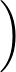+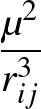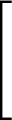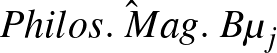-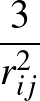(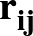)()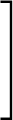.

• STOCKSPIN x n: Activates checking for alignment of dipoles with the z axis in the Stockmayer potential. Such alignment makes the polar angle φ for the dipole redundant, leading to extra zero Hessian eigenvalues and associated problems. If alignment is detected at any step in an optimisation then the orientation of the cluster is randomised and a warning is printed. x is the amount by which | cosθ| may differ from 1 for the dipole to be counted as aligned with the z axis. n is the maximum number of attempts to remove aligned dipoles by random rotation. Values of 10-4 and 10, respectively, are a sensible starting point.

• STOPDIST x: in a CONNECT run, stop as soon as the distance between the initial (x> 0) or final (x< 0) minimum and the furthest connected minimum exceeds | x|.

• STOPFIRST: in a CONNECT run, stop as soon as the initial minimum has a transition state connection.

• SUMMARY n: prints a summary of the steps and derivatives every n steps. The default is n = 20.

• SYMCUT x: specifies the RMS force below which the point group symmetry subroutine will be called. The default is x=0.001.

• TAG num massfac: allows atoms to be tagged. Atom number num has its mass increased by a factor massfac for point group symmetry assignment only. To tag more than one atom use additional TAG lines in odata.

• TD: add a tetrahedral field of strength x to the potential.

• TIMELIMIT x: OPTIM will stop if the accumulated cpu time exceeds x seconds. The default value is infinity.

• TOLD x: sets the initial distance tolerance for points to be considered the same after rotations and reflections in point group determination. The default is 0.0001.

• TOLE x: sets the initial tolerance for eigenvalues of the inertia tensor to be considered equivalent in point group determination. The default is 0.0001.

• TOMEGA: includes omega angles in the TWISTDIHE list.

• TOSI A++ A A+- ρ: specifies a Born-Mayer potential with the parameters indicated (see §4).

• TOSIC6 c6pp c6mm c6pm: specifies additional C6 dispersion coefficients for the TOSI potential. c6pp, c6mm, and c6pm should be the values of the C6 parameters between positive ions, negative ions and positive and negative ions in atomic units (see §4).

• TOSIPOL alphap alpham damp: specifies cation and anion polarisabilities in atomic units and a damping parameter for the TOSI potential (see §4).

• TRAD x: sets the trust radius (see §8). The default value is 2.0.

• TRAP k n: general trapped ion potential coded by Ersin Yurtsever. The form of the potential is

V =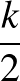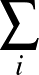rin +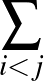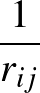,

where ri is the distance of ion i from the origin and rij is the distance between ions i and j.

• TSIDECHAIN: includes sidechain angles in the TWISTDIHE list.

• TSPLITTING: Tunnelling splittings of the vibrational ground state level following . Every time a rate is calculated, the tunnelling splitting is calculated as well. Tunnelling splittings only make sense for symmetric molecules and barriers.

• TTM3: specifies Xantheas' TTM3-F water potential, coded by Jeremy Richardson. The atom order must be O1, O2,..., H1a, H1b, H2a, H2b,.... The energy is in kcal/mol and the distance unit is Å. Hydrogens are NOT permutable between different oxygens.

• TWIST n1 n2 n3 n4 f ref: adds a twist potential corresponding to the torsional angle for atoms n1, n2, n3 and n4. The functional form is Vtwist(φ) = f sin(φ - φref. phiref and the angular displacement are set to the range - π to π, but with the sin function dependence this should now make no difference. Setting φref to the angle for the global minimum or native state might be convenient.

• TWISTDIHE random xpert: twist phi/psi dihedral angle selected from an array of safely twistable dihedrals using the random number random between 0 and 1 by xpert degrees before starting optimisation. This keyword is no longer designed to be user specified, but is used by PATHSAMPLE.

• TWISTTYPE n: integer n specifies the type of dihedral angle twisting used to guess transition states in guessts for CHARMM and UNRES. The recommended value is n=5. See also NORANDOM.

• TWOENDS fstart finc ntwo rmstwo ntwoiter twoeval: obsolete: specifies a double-ended transition state search. fstart is the initial force constant pulling the coordinates towards the final geometry which is read from a file named final. finc is the increment for the force constant after each cycle. ntwo is the maximum number of minimisation steps taken after each increment of the force constant, and rmstwo is the convergence condition on the RMS force for these minimisations. ntwoiter is the total number of force constant increments allowed and twoeval is the value of the smallest Hessian eigenvalue below which the program will shift to a transition state search.

• UACHIRAL: MUST be included when using ff03ua, the AMBER united atom forcefield. It ensures the correct impropers are used to define sidechain chirality when HB hydrogen is missing.

• UNRES: specifies the UNRES potential, which requires an UNOPTIM executable. An auxiliary file coords is required. UNRES must be the last OPTIM directive in the odata file. The remaining content of odata consists of UNRES keywords and setup information.

• UPDATES mupdate1 mupdate2 mupdate3 mupdate4: specifies the number of updates in the LBFGS routine before resetting. mupdate1 is used for energy minimisation, mupdate2 is currently ignored by mind, mupdate3 is used in Rayleigh-Ritz eigenvalue calculations, and mupdate4 is used in NEB and DNEB optimisation. mupdate5 is used in string method optimisation. The default value is 4 for all five parameters.

• USEDIAG n: allows different approximations to be used for the eigenvalue in a Rayleigh-Ritz BFGSTS calculation. n = 1 specifies the second order central differences method. n = 2 is the same accuracy, but uses a formula that minimises the rounding error, which may work better for numerically large values of the energy and is the default behaviour. n = 3 specifies the first order forward finite differences method which is less accurate, but requires one fewer potential call per iteration.

• USEEV n : specifies the number of non-zero (lowest) eigenvalues and eigenvectors to be calculated by LAPACK routine DSYEVR in efol.f, bfgsts.f or hybridmin.f. If this value is not specified than full diagonalisation is performed in efol.f using LAPACK routine DSYEV, while the default is a single eigenvalue/eigenvector for bfgsts.f and hybridmin.f.

• VALUES n: prints the Hessian eigenvalues every n steps. The default is n=20.

• VARIABLES: optimises a general function (which must be specified in potential. The initial values of the variables are read one per line after the POINTS keyword in odata.

• VARSTEPOPT: Allows instanton optimization with a variable step size distributino of the beads as described in .

• VASP vaspjob: tells OPTIM to use the VASP executable to calculate energies and gradients. The string vaspjob specifies the run command for VASP, for example on CSD3:
VASP 'mpirun -ppn 16 -np 16 /home/bk393/APPS/vasp.5.4.4/with-VTST/bin/vasp_std > vasp.out'
The setup has been changed so that both start and finish coordinates are read from files in the VASP POSCAR format. The coordinates can be Cartesian or fractional (Direct keyword in POSCAR). PPOSCAR files can be visualised directly with ase (Atomic Simulation Environment). To access this program on volkhan:
which assumes that /.input/bin is in the current $PATH environment variable. • VECTORS n: prints the Hessian eigenvectors every n steps. Eigenvector printing is turned off by default. • WARRANTY: print warranty information. • WELCH A++ A A+- ρ Q+ Q- α+ α-: specifies a Welch binary salt potential with the parameters indicated (see §4). • XTB system runparams: provides an interface to the xtb program; see the documentation at xtb-docs.readthedocs.io/en/latest/contents.html. system is a string with a name for the job; runparams is a string with options for the xtb executable, which must be in the current path. For example: XTB water21 '–acc 0.1 –gfn2 -P 8' Note: OPTIM assumes that the coordinates are in Å, which needs a$coords angs directive in the xtb input file. There is a conversion factor in the gradient, to account for xtb gradient printing in hartree/bohr even when the coordinates are in Å.